# EP3045934A1 - Radar device, vehicle, and moving-body-speed detection method - Google Patents

## Info

Publication number
EP3045934A1
EP3045934A1 EP14844250.2A EP14844250A EP3045934A1 EP 3045934 A1 EP3045934 A1 EP 3045934A1 EP 14844250 A EP14844250 A EP 14844250A EP 3045934 A1 EP3045934 A1 EP 3045934A1
Authority
EP
European Patent Office
Prior art keywords
unit
transmission
θ
signals
Prior art date
Legal status (The legal status is an assumption and is not a legal conclusion. Google has not performed a legal analysis and makes no representation as to the accuracy of the status listed.)
Pending
Application number
EP14844250.2A
Other languages
German (de)
French (fr)
Other versions
EP3045934A4 (en
Inventor
Takaaki Kishigami
Hirofumi Nishimura
Current Assignee (The listed assignees may be inaccurate. Google has not performed a legal analysis and makes no representation or warranty as to the accuracy of the list.)
Panasonic Corp
Original Assignee
Panasonic Corp
Priority date (The priority date is an assumption and is not a legal conclusion. Google has not performed a legal analysis and makes no representation as to the accuracy of the date listed.)
Filing date
Publication date
Priority to JP2013189380 priority Critical
Application filed by Panasonic Corp filed Critical Panasonic Corp
Priority to PCT/JP2014/003790 priority patent/WO2015037173A1/en
Publication of EP3045934A1 publication Critical patent/EP3045934A1/en
Publication of EP3045934A4 publication Critical patent/EP3045934A4/en
Pending legal-status Critical Current

• 230000001427 coherent Effects 0.000 claims description 62
• 230000000051 modifying Effects 0.000 claims description 36
• 230000000875 corresponding Effects 0.000 claims description 26
• 239000000284 extracts Substances 0.000 claims description 3
• 230000000694 effects Effects 0.000 description 5
• 238000005070 sampling Methods 0.000 description 5
• 238000000034 methods Methods 0.000 description 2
• 239000000203 mixtures Substances 0.000 description 2
• 238000005516 engineering processes Methods 0.000 description 1

## Images

•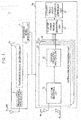•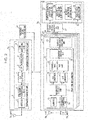•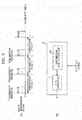•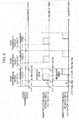•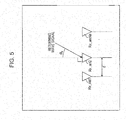•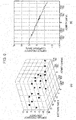•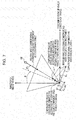•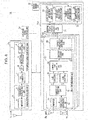•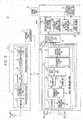•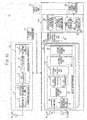•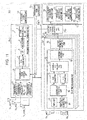•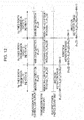•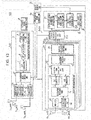•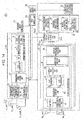•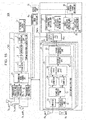•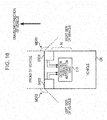••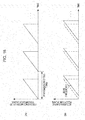•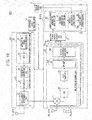•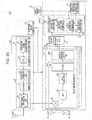•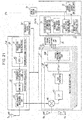•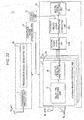•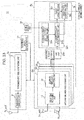•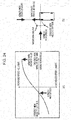•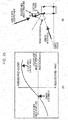•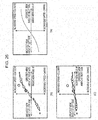•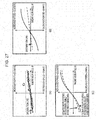•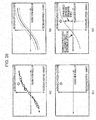•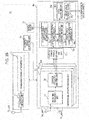•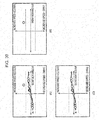•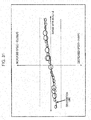•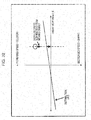## Classifications

• GPHYSICS
• G01MEASURING; TESTING
• G01S13/00Systems using the reflection or reradiation of radio waves, e.g. radar systems; Analogous systems using reflection or reradiation of waves whose nature or wavelength is irrelevant or unspecified
• G01S13/02Systems using reflection of radio waves, e.g. primary radar systems; Analogous systems
• G01S13/50Systems of measurement based on relative movement of target
• G01S13/58Velocity or trajectory determination systems; Sense-of-movement determination systems
• G01S13/60Velocity or trajectory determination systems; Sense-of-movement determination systems wherein the transmitter and receiver are mounted on the moving object, e.g. for determining ground speed, drift angle, ground track
• GPHYSICS
• G01MEASURING; TESTING
• G01S13/00Systems using the reflection or reradiation of radio waves, e.g. radar systems; Analogous systems using reflection or reradiation of waves whose nature or wavelength is irrelevant or unspecified
• G01S13/02Systems using reflection of radio waves, e.g. primary radar systems; Analogous systems
• G01S13/06Systems determining position data of a target
• G01S13/08Systems for measuring distance only
• G01S13/10Systems for measuring distance only using transmission of interrupted pulse modulated waves
• G01S13/26Systems for measuring distance only using transmission of interrupted pulse modulated waves wherein the transmitted pulses use a frequency- or phase-modulated carrier wave
• GPHYSICS
• G01MEASURING; TESTING
• G01S13/00Systems using the reflection or reradiation of radio waves, e.g. radar systems; Analogous systems using reflection or reradiation of waves whose nature or wavelength is irrelevant or unspecified
• G01S13/02Systems using reflection of radio waves, e.g. primary radar systems; Analogous systems
• G01S13/06Systems determining position data of a target
• G01S13/08Systems for measuring distance only
• G01S13/32Systems for measuring distance only using transmission of continuous unmodulated waves, amplitude-, frequency- or phase-modulated waves
• G01S13/34Systems for measuring distance only using transmission of continuous unmodulated waves, amplitude-, frequency- or phase-modulated waves using transmission of frequency-modulated waves and the received signal, or a signal derived therefrom, being heterodyned with a locally-generated signal related to the contemporaneous transmitted signal to give a beat-frequency signal
• GPHYSICS
• G01MEASURING; TESTING
• G01S13/00Systems using the reflection or reradiation of radio waves, e.g. radar systems; Analogous systems using reflection or reradiation of waves whose nature or wavelength is irrelevant or unspecified
• G01S13/02Systems using reflection of radio waves, e.g. primary radar systems; Analogous systems
• G01S13/06Systems determining position data of a target
• G01S13/42Simultaneous measurement of distance and other co-ordinates
• GPHYSICS
• G01MEASURING; TESTING
• G01S13/00Systems using the reflection or reradiation of radio waves, e.g. radar systems; Analogous systems using reflection or reradiation of waves whose nature or wavelength is irrelevant or unspecified
• G01S13/93Radar or analogous systems specially adapted for specific applications for anti-collision purposes
• G01S13/931Radar or analogous systems specially adapted for specific applications for anti-collision purposes of land vehicles
• GPHYSICS
• G01MEASURING; TESTING
• G01S3/00Direction-finders for determining the direction from which infrasonic, sonic, ultrasonic, or electromagnetic waves, or particle emission, not having a directional significance, are being received
• G01S3/02Direction-finders for determining the direction from which infrasonic, sonic, ultrasonic, or electromagnetic waves, or particle emission, not having a directional significance, are being received using radio waves
• G01S3/14Systems for determining direction or deviation from predetermined direction
• G01S3/46Systems for determining direction or deviation from predetermined direction using antennas spaced apart and measuring phase or time difference between signals therefrom, i.e. path-difference systems
• GPHYSICS
• G01MEASURING; TESTING
• G01S7/00Details of systems according to groups G01S13/00, G01S15/00, G01S17/00
• G01S7/02Details of systems according to groups G01S13/00, G01S15/00, G01S17/00 of systems according to group G01S13/00
• G01S7/28Details of pulse systems

## Abstract

A radar device mounted to a moving object includes a radar transmission unit that transmits a radio-frequency radar transmission signal from a transmission antenna, at each transmission cycle, and a radar reception unit that receives a returning signal that is the radar transmission signal reflected off of a target, by a plurality of reception antennas. The radar reception unit includes a plurality of antenna system processing units that perform correlation processing of returning signals and the radar transmission signals, and generate correlation signals including arrival delay information of the returning signals, an electric power profile generating unit that generates an electric power profile for each arrival direction of the returning signals and Doppler frequency component, using the correlation signals acquired by the plurality of reception antennas, and a stationary object group distribution generating unit that, based on the electric power profile of the returning signals, obtains a first distribution of a Doppler frequency component of a stationary object group including a plurality of stationary objects in the perimeter of the moving object, for each azimuth angle.

## Description

Technical Field
• The present disclosure relates to a radar device installed in a moving object (e.g., a vehicle), the moving object in which the radar device is installed, and a moving object speed detection method of detecting a speed of travel of the moving object.
• Background Art
• There are known radar devices which receive returning signals reflected off of a target at an antenna array, and measure the phase difference of the reception signals received by reception antenna elements, thereby estimating a direction of arrival of returning signals from the target over a wide angle range.
• Examples of prior art relating to installing a radar device capable of wide angle range measurement in a moving object (e.g., a vehicle), and detecting the position and relative speed of a target in the perimeter, include PTL 1 and PTL 2.
• A target speed calculating device shown in PTL 1 calculates, based on a radar device measuring relative speed Vr and an azimuth θr as to a target vehicle ahead of a subject vehicle, a vehicular speed sensor that detects vehicular speed Vs of the subject vehicle, and an azimuth θr of the object (target) which is to be measured by the radar device, an angle θ between the direction of travel of the vehicle and the azimuth of the object (= θr + θs). The speed Vo of the target is corrected to Vo = Vs x cos(θr + θs) - Vr at a computing unit, based on the relative speed Vr, speed Vs of the vehicle and the angle θ. Note that the angle θs between the direction of travel of the subject vehicle and the axle is calculated one combination out of the combinations "rudder angle sensor, vehicular speed sensor, and yaw rate sensor" and "vehicular speed sensor and side acceleration sensor".
• Also, a vehicular moving state detection device shown in PTL 2 uses multiple side monitoring radar devices to identify stationary objects. Specifically, based on detection values of azimuth and relative speed for each electromagnetic wave reflection part, the vehicular moving state detection device uses an azimuth ϕ of an electromagnetic wave reflection part situated closest to the subject vehicle out of the electromagnetic wave reflection parts, and a relative speed Vd0, so as to correct the measurement value of relative speed Vd0 at the electromagnetic wave reflection part to Vd = Vd0 × cosϕ. In a case where the relative speed Vd after correction is equivalent to the detection value is equivalent to the detected value V0 of the onboard speedometer, an aggregate of electromagnetic wave reflection parts is identified as being a stationary object. The azimuth ϕ of the electromagnetic wave reflection part is an azimuth with reference to the frontal direction of the subject vehicle (ϕ = 0).
• Citation List Patent Literature
• PTL 1: Japanese Unexamined Patent Application Publication No. 2004-177289
• PTL 2: Japanese Unexamined Patent Application Publication No. 2010-043960
Summary of Invention Technical Problem
• Output values of a vehicular speed sensor installed onboard beforehand are used as the traveling speed (vehicular speed) of the subject vehicle, to calculate the corrected value Vo of the speed of the target in PTL 1, and the corrected value Vd of the relative speed of the electromagnetic wave reflection part in PTL 2, described above. The measurement error of the vehicular speed sensor tends to become larger the faster the traveling speed of the vehicle is, or the greater the acceleration is, which is problematic in that the detection precision of relative speed of a target (or electromagnetic wave reflection part) is insufficient.
• In order to solve the above-described conventional problems, the present disclosure provides a radar device that suppresses measurement error in traveling speed of a moving object in which the radar device is mounted (e.g., a vehicle), and improves detection precision of the relative speed of the target. Solution to Problem
• The preset disclosure is a radar device mounted to a moving object. The radar device includes a radar transmission unit that transmits a radio-frequency radar transmission signal from a transmission antenna, at each transmission cycle, and a radar reception unit that receives a returning signal that is the radar transmission signal reflected off of a target, by a plurality of reception antennas. The radar reception unit includes a plurality of antenna system processing units that perform correlation processing of returning signals and the radar transmission signals, and generate correlation signals including arrival delay information of the returning signals, an electric power profile generating unit that generates an electric power profile for each arrival direction of the returning signals and Doppler frequency component, using the correlation signals acquired by the plurality of reception antennas, and a stationary object group distribution generating unit that, based on the electric power profile of the returning signals, obtains a first distribution of a Doppler frequency component of a stationary object group including a plurality of stationary objects in the perimeter of the moving object, for each azimuth angle.
• The present disclosure also is a vehicle, including: a first sector radar, of which a right front area to a right side area of the vehicle is a first detection range; a second sector radar, of which a left front area to a left side area of the vehicle is a second detection range; and a detection result integration unit that corrects the relative speed of the target at the first sector radar or the second sector radar, in accordance with difference between a first estimation value of traveling speed of the vehicle at the first sector radar, and a second estimation value of traveling speed of the vehicle at the second sector radar.
• The present disclosure also is a moving object speed detecting method in a radar device mounted to the moving object. The method includes: transmitting a radio-frequency radar transmission signal from a transmission antenna, at each transmission cycle; receiving a returning signal that is the radar transmission signal reflected off of a target, by a plurality of reception antennas; performing correlation processing of returning signals and the radar transmission signals, and generating correlation signals including arrival delay information of the returning signals; generating an electric power profile for each arrival direction of the returning signals and Doppler frequency component, using the correlation signals acquired by the plurality of reception antennas; obtaining, based on the electric power profile of the returning signals, a first distribution of a Doppler frequency component of a stationary object group including a plurality of stationary objects in the perimeter of the moving object, for each azimuth angle; and estimating the traveling speed of the moving object, based on the first distribution of Doppler frequency components of the stationary object group, at each azimuth angle. Advantageous Effects of Invention
• According to the present disclosure, measurement error in traveling speed of a moving object in which the radar device is mounted (e.g., a vehicle) can be suppressed, and detection precision of the relative speed of the target improved. Brief Description of Drawings
• [Fig. 1] Fig. 1 is a block diagram illustrating the internal configuration of a radar device according to a first embodiment in brief.
• [Fig. 2] Fig. 2 is a block diagram illustrating the internal configuration of the radar device according to the first embodiment in detail.
• [Fig. 3] (A) is a diagram illustrating the relationship between transmission periods and transmission cycles of radar transmission signals, and (B) is a block diagram illustrating the internal configuration of another transmission signal generating unit.
• [Fig. 4] Fig. 4 is a diagram illustrating the relationship between radar transmission signals, radar reception signals at delay time τ1, and radar reception signals at delay time τ2.
• [Fig. 5] Fig. 5 is an explanatory diagram illustrating the relationship between the placement and azimuth angle θu of reception antenna elements making up an array antenna.
• [Fig. 6] (A) is a graph illustrating an output example of an electric power profile Fout(k, fs, θu, w) at each distance / azimuth angle / Doppler frequency component, and (B) is a graph illustrating an example of an approximated line of Doppler frequency component f_Nmaxu, w) in which the fs count value N_CNmaxu, w) in Fig. 6(A), where electric power profile Fout(k, fs, θu, w) > 0, is greatest.
• [Fig. 7] Fig. 7 is an explanatory diagram of a coordinates system as to the frontal direction (direction of travel) of a vehicle, in a case where the array antenna illustrated in Fig. 5 has been mounted to a vehicle CR.
• [Fig. 8] Fig. 8 is a block diagram illustrating the internal configuration of the radar device according to a second embodiment in detail.
• [Fig. 9] Fig. 9 is a block diagram illustrating the internal configuration of the radar device according to a third embodiment in detail.
• [Fig. 10] Fig. 10 is a block diagram illustrating the internal configuration of the radar device according to a fourth embodiment in detail.
• [Fig. 11] Fig. 11 is a block diagram illustrating the internal configuration of the radar device according to a fifth embodiment in detail.
• [Fig. 12] Fig. 12 is a diagram illustrating the relationship between the outputs of a transmission beam control unit, transmission beam forming unit, and electric power profile generating unit, and transmission cycle.
• [Fig. 13] Fig. 13 is a block diagram illustrating the internal configuration of a radar device according to a first modification of the fifth embodiment in detail.
• [Fig. 14] Fig. 14 is a block diagram illustrating the internal configuration of a radar device according to a second modification of the fifth embodiment in detail.
• [Fig. 15] Fig. 15 is a block diagram illustrating the internal configuration of a radar device according to a third modification of the fifth embodiment in detail.
• [Fig. 16] Fig. 16 is a block diagram illustrating the internal configuration of the radar device according to a sixth embodiment in detail.
• [Fig. 17] Fig. 17 is a block diagram illustrating the internal configuration of the radar device according to a seventh embodiment in detail.
• [Fig. 18] (A) is a diagram illustrating the relationship between frequency modulation transmission signals and transmission cycle, and (B) is a diagram illustrating received returning signals from a target of the frequency modulation transmission signals illustrated in Fig. 18(A).
• [Fig. 19] Fig. 19 is a block diagram illustrating the internal configuration of a radar device according to a first modification of the seventh embodiment in detail.
• [Fig. 20] Fig. 20 is a block diagram illustrating the internal configuration of a radar device according to a second modification of the seventh embodiment in detail.
• [Fig. 21] Fig. 21 is a block diagram illustrating the internal configuration of a radar device according to a third modification of the seventh embodiment in detail.
• [Fig. 22] Fig. 22 is a block diagram illustrating the internal configuration of a radar device according to an eighth embodiment in detail.
• [Fig. 23] Fig. 23 is a block diagram illustrating the internal configuration of a stationary object group distribution generating unit and moving target detecting unit according to the eighth embodiment.
• [Fig. 24] (A) is a diagram illustrating the relationship between relative speed (Doppler speed) and azimuth angle (second distribution) estimated in a case where the vehicle is in a state of proceeding straight and the radar device is disposed on the left front of the vehicle, and (B) is a diagram illustrating the relationship between a radar view angle θ of the radar device disposed on the vehicle in a state of proceeding straight, traveling direction θr of vehicle, azimuth at which an estimated relative speed (Doppler speed) Ve becomes greatest, and azimuth at which the estimated relative speed Ve becomes 0.
• [Fig. 25] (A) is a diagram illustrating the relationship between relative speed Ve and azimuth angle θ estimated in a case where the vehicle is in a state of turning left and a radar device 1 H is disposed on the left front of the vehicle, and (B) is a diagram illustrating the relationship between a radar view angle θ of the radar device 1 H disposed on the vehicle in a state of turning left, traveling direction θr of vehicle, azimuth at which a relative speed Ve becomes greatest, and azimuth at which the relative speed Ve becomes 0.
• [Fig. 26] (A) is a diagram illustrating the relationship between the radar view angle θ obtained from an electric power profile and Doppler speed, (B) is a diagram illustrating the relationship between the radar view angle θ estimated from the traveling state of the vehicle and relative speed as to a stationary object, and (C) is a diagram illustrating distribution of electric power profile after performing correction processing using Fig. 26(B).
• [Fig. 27] (A) is a diagram illustrating the relationship between the distribution of electric power profile after performing correction processing and a distribution line of stationary objects, (B) is a diagram illustrating the relationship between the Doppler speed of estimated stationary objects and radar view angle, and Fig. 27(C) is a diagram illustrating the relationship between the estimated Doppler speed of stationary objects after correction processing and radar view angle.
• [Fig. 28] (A) is a diagram illustrating the relationship between the radar view angle θ obtained from an electric power profile and Doppler speed, (B) is a diagram where a margin (dotted lines) has been added in the speed direction to the Doppler speed (solid line) of the stationary object estimated from the traveling state of the vehicle, (C) is a diagram illustrating an electric power profile distribution after having removed the electric power profile included in the margin in Fig. 28(B) from the electric power profile in Fig. 28(A), and (D) is a diagram illustrating correction of speed error of a moving object using a distribution line.
• [Fig. 29] Fig. 29 is a block diagram illustrating the configuration of a radar device 1J according to the eighth embodiment.
• [Fig. 30] (A) is a diagram where a margin has been set to the electric power profile after Doppler speed correction in Fig. 26(C), (B) is a diagram where an electric power profile outside of the margin has been extracted as a moving object, and (C) is a diagram where an electric power profile inside the margin has been extracted as a stationary object.
• [Fig. 31] Fig. 31 is a diagram illustrating a distribution line obtained as to an electric power profile extracted as a moving object, in a first modification of the eighth embodiment.
• [Fig. 32] Fig. 32 is a diagram illustrating the relationship between the distribution line obtained in Fig. 31, and correction of the speed of the electric power profile extracted as a moving object, in the first modification of the eighth embodiment.
Description of Embodiments
• (Background Leading to the Content of the Embodiments of the Radar Device, Vehicle, and Moving Object Speed Detection Method According to the Present Disclosure)
• First, the background leading to the content of the embodiments of the radar device, vehicle, and moving object speed detection method according to the present disclosure will be described, before describing the content of the embodiments of the radar device, vehicle, and moving object speed detection method according to the present disclosure.
• A vehicular speed measurement value Vc acquired from a vehicular speed sensor installed in the vehicle beforehand, in the above-described PTLs 1 and 2 includes vehicular speed measurement error due to the state of the vehicle (e.g., the state of wear of the tires of the vehicle), the state of travel of the vehicle (e.g., the state of sideslip of the vehicle when turning right and left), and the operating state of the vehicular speed sensor (e.g., delay in detection). Also, the faster the traveling speed of the vehicle is, and the greater the acceleration is, the greater the vehicular speed measurement error tends to be. That is to say, the vehicular speed measurement value Vc acquired from the vehicular speed sensor installed in the vehicle beforehand in the above-described PTLs 1 and 2 includes error as to the actual vehicular speed, so the detection precision of relative speed of the target by the radar device deteriorates.
• In a case where there is time delay until the vehicular speed measurement value Vc being obtained, the detection processing of the relative speed of the target by the radar device is delayed. In a case where the vehicle is accelerating, the vehicular speed charges, so the greater the difference between the time till the vehicular speed sensor detects the vehicular speed measurement value Vc and the time till the radar device detects the target is, the greater the deterioration in detection precision of the relative speed of the target by the radar device is.
• Also, in a case where the vehicle is turning at an intersection, for example, or driving along a gentle curve, i.e., in a case where the vehicle is curving, Doppler frequency component shift occurs in the range (distance) direction of the radar device, in accordance with the moment of the vehicle. Accordingly, difference occurs between the Doppler frequency component estimated using the vehicular speed measurement value Vc acquired from the vehicular speed measurement sensor and the actual (Doppler frequency component, so deterioration occurs in detection precision of the relative speed of the target by the radar device.
• Note that when the vehicle is traveling along a straight road, the driver performs fine adjustments using the driving wheel to stay in the lane. However, the radius of curvature of the movement locus of the vehicle due to the fine adjustments by the driving wheel is sufficiently large to where movement of the vehicle due to the fine adjustments using the driving wheel to stay in the lane is determined to be the vehicle traveling straight.
• Accordingly, examples of a radar device and moving object speed detection method where detection precision of the relative speed of a target is improved, will be described in the following embodiments.
• Embodiments of the radar device and moving object speed detection method according to the present disclosure will be described below with reference to the drawings.
• (First Embodiment)
• The configuration and operations of a radar device 1 according to a first embodiment will be described with reference to Fig. 1 through Fig. 5. Fig. 1 is a block diagram illustrating the internal configuration of the radar device 1 according to the first embodiment in brief. Fig. 2 is a block diagram illustrating the internal configuration of the radar device 1 according to the first embodiment in detail. Fig. 3(A) is a diagram illustrating the relationship between transmission periods and transmission cycles of radar transmission signals. Fig. 3(B) is a block diagram illustrating the internal configuration of another transmission signal generating unit.
• The radar device 1 is installed in a moving object which travels straight or travels curving following a direction of travel (e.g., vehicle CR. The same hereinafter.) However, the moving object to which the radar device 1 is installed is not restricted to the vehicle CR, and includes motorcycles and autonomous robots, for example.
• The radar device 1 transmits radio-frequency radar transmission signals generated at a radar transmission unit Tx from a transmission antenna Tx_ant1. The radar device 1 receives the radar transmission signals reflected off of a target (omitted from illustration) at an array antenna (e.g., four reception antennas Rx_ant1 through Rx_ant4 illustrated in Fig. 1) as returning signals. The radar device 1 uses the results of having performed signal processing on the returning signals received by the four reception antennas Rx_ant1 through Rx_ant4 to detect whether or not there is a target, and further estimates a vehicular speed vc of the vehicle CR to which the radar device 1 is mounted, and a relative speed vd of the target.
• Note that the target is an object of detection by the radar device 1, including automobile and people for example, which is the same in the following embodiments hereinafter. Note that the reception antennas Rx_ant1 through Rx_ant4 may be reception antenna elements. The array antenna of the radar device 1 is not restricted to the four reception antennas Rx_ant1 through Rx_ant4.
• First, description will be made in brief of the configurations of the parts of the radar device 1.
• The radar device 1 illustrated in Fig. 1 includes a reference signal generating unit Lo, the radar transmission unit Tx, and a radar reception unit Rx. The radar transmission unit Tx includes a transmission signal generating unit 2, and a transmission wireless unit 3 to which the transmission antenna Tx_ant1 is connected.
• The reference signal generating unit Lo is connected to the radar transmission unit Tx and the radar reception unit Rx. The reference signal generating unit Lo supplies the radar transmission unit Tx and radar reception unit Rx with reference signals, thereby synchronizing the radar transmission unit Tx and the radar reception unit Rx.
• The radar reception unit Rx includes, for example, four antenna system processing units D1, D2, D3, and D4, an electric power profile generating unit 21, and a stationary object group distribution generating unit 22. While the radar reception unit Rx illustrated in Fig. 1 includes the four antenna system processing units D1 through D4 in accordance with the number of reception antennas, for example, the number of the antenna system processing units is not restricted to four, and may be two or more. Note that the antenna system processing units D1 through D4 have the same configuration, so the antenna system processing unit D1 will be exemplarily described in the following embodiments.
• The antenna system processing unit D1 includes a delay time measuring unit 12 to which the reception antenna Rx_ant1 is connected, and a Doppler frequency analyzing unit 20.
• Next, the operations of the parts of the radar transmission unit Tx and radar reception unit Rx illustrated in Fig. 1 will be described in detail.
• The transmission signal generating unit 2 generates a transmission reference clock signal based on the reference signal generated by the reference signal generating unit Lo, where the reference signal has been multiplied to a predetermined multiple. The parts of the transmission signal generating unit 2 operate based on the transmission reference clock signal.
• The baseband transmission signal generated by the transmission signal generating unit 2 may be a coded pulse such as illustrated in Fig. 3(A) or a chirp pulse illustrated in Fig. 18, being repeatedly transmitted according to a predetermined transmission frequency.
• The transmission wireless unit 3 upconverts the transmission signal generated by the transmission signal generating unit 2 to generate a carrier frequency band (e.g., millimeter wave band) radar transmission signal.
• The transmission antenna Ant-Tx1 emits the radar transmission signal generated by the transmission wireless unit 3 into space.
• The reception antenna Rx_ant1 receives returning signals which are the radar transmission signals transmitted by the radar transmission unit Tx and reflected off of the target. The radio-frequency radar reception signals which the reception antenna Rx_ant1 has received are input to the delay time measuring unit 12.
• The delay time measuring unit 12 downconverts the radar reception signals which the reception antenna Rx_ant1 has received, thereby generating baseband reception signals.
• The delay time measuring unit 12 performs correlation calculating between the reception signals input from the reception antenna Rx_ant and the transmission signals at each transmission cycle, and measures the reception delay time of the returning signals reflected off of the target, i.e., a delay time τ from the transmission start time of the radar transmission signal.
• The Doppler frequency analyzing unit 20 performs coherent addition processing on the output of each reception delay time, using phase shift according to multiple Doppler frequency components fsΔθ as correction coefficients. Accordingly, the Doppler frequency analyzing unit 20 outputs coherent addition processing results of multiple Doppler frequency components at each arrival delay time. Note that the output of each correction coefficient and reception delay time is obtained at each transmission cycle or an integer multiple thereof.
• The electric power profile generating unit 21 is input with the coherent addition calculation processing results of multiple Doppler frequency components each arrival delay time, and configures a correlation vector h. Note that the coherent addition calculation processing results are input from the antenna system processing units D1 through D4 at each transmission cycle or an integer multiple thereof.
• In a case where the correlation vector h satisfies predetermined conditions, the electric power profile generating unit 21 obtains electric power profile Fout for each distance / azimuth angle / Doppler frequency component in returning signals reflected off of the target, by computing the square of the inner product of the correlation vector h and a directional vector "a" stored beforehand.
• On the other hand, in a case where the correlation vector h does not satisfy predetermined conditions, the electric power profile generating unit 21 obtains the electric power profile Fout for each distance / azimuth angle / Doppler frequency component as 0 (zero).
• The stationary object group distribution generating unit 22 obtains the distribution of Doppler frequency components of a stationary object group including multiple stationary objects in the perimeter, for each azimuth angle with the vehicle CR as a reference, based on the electric power profile Fout (electric power profile of the returning signals) input from the electric power profile generating unit 21.
• The stationary object group distribution generating unit 22 analyzes the Doppler frequency distribution for each azimuth angle, based on the electric power profile Fout obtained from the electric power profile generating unit 21 (See Fig. 6(A)). Fig. 6(A) is a graph illustrating an output example of the electric power profile Fout for each distance / azimuth angle / Doppler frequency component. Fig. 6(A) shows the electric power profile Fout obtained from the electric power profile generating unit 21 for each discrete time k, azimuth (angle) [radian], and Doppler frequency component fs.
• The stationary object group distribution generating unit 22 obtains an approximation curve (distribution) of Doppler frequency components of the stationary object group, for each azimuth angle, as the distribution of Doppler frequency components of the stationary object group for each azimuth angle (see Fig. 6(B)).
• Next, the configuration of the parts of the radar transmission unit Tx will be described in detail with reference to Fig. 2. The radar transmission unit Tx illustrated in Fig. 2 includes the transmission signal generating unit 2, and the transmission wireless unit 3 to which the transmission antenna Tx_ant1 is connected.
• The transmission signal generating unit 2 includes a code generating unit 4, a modulator 5, an LPF (Low Pass Filter) 6. and a D/A (Digital Analog) converting unit 7. The LPF 6 in Fig. 2 may be disposed externally from the transmission signal generating unit 2. The output of the LPF 6 is input to the D/A converter 7. The transmission wireless unit 3 includes a frequency converting unit 8 and an amplifier 9.
• Now, a case of using coded pulses in the transmission signal generating unit 2 will be described.
• First, the coded pulses illustrated in Fig. 3(A) will be described. In a transmission period Tw [seconds] of a transmission cycle Tr, for example, modulation is performed using No [count] samples of transmission reference clock signals per code of a code sequence Cn having a code length L. Here, n is 1 through L (>0). L (an integer) represents the code length of the code sequence Cn.
• The sampling rate at the transmission signal generating unit 2 is (No × L) / Tw, the transmission signal generating unit 2 performing modulation using Nr (= No × L) [count] samples in a transmission period Tw [seconds] of a transmission cycle Tr. The transmission signal generating unit 2 performing modulation using Nu [count] samples in a period without signal (Tr - Tw) [seconds] of a transmission cycle Tr.
• The transmission signal generating unit 2 periodically generates baseband transmission signals r(k, M) illustrated in Expression (1), by modulation of the code sequence Cn of the code length L. j is an imaginary unit satisfying j2 = -1, time k is a discrete time with the start timing of the transmission cycle Tr as a reference (k = 1), k being a discrete value from 1 to (Nr + Nu), serving as a point-in-time representing the timing of generating the transmission signals (sample timing).
• M represents the ordinal of the transmission cycle Tr of radar transmission signals. Transmission signal r(k, M) represents a transmission signal at discrete time k in at the M'th transmission cycle Tr, being the addition results of in-phase signal component l(k, M), and orthogonal signal component Q(k, M) multiplied by the imaginary unit j (see Expression (1)).
[Math. 1] $r k M = I k M + jQ k M$
• The code generating unit 4 generates transmission code of the code sequence Cn of code length L at each transmission cycle Tr. The elements of the code sequence Cn are configured using the two values of [-1, 1], or the four values of [1, -1, j, -j], for example. This transmission code is preferably code including at least one of a code sequence making up a pair of complementary codes, a Barker code sequence, a PN (Pseudorandom Noise) code, a Golay code sequence, an M-sequence code, and a code sequence making up a Spano code, so that the radar reception unit Rx will exhibit low side lobe characteristics. The code generating unit 4 outputs the transmission code of the generated code sequence Cn to the modulator 5. Hereinafter, "transmission code of the code sequence Cn" will be written as "transmission code Cn" for sake of convenience.
• In a case of generating a pair of complementary codes (e.g., Golay code sequence, Spano code sequence) as the transmission code Cn, the code generating unit 4 generates each of transmission codes Pn and Qn which are alternately a pair each transmission cycle, using two transmission cycles (2Tr). That is to say, at the M'th transmission cycle, the code generating unit 4 generates and outputs to the modulator 5 the one transmission code Pn making up the pair of complementary codes, and at the subsequent (M+1)'th transmission cycle, generates and outputs to the modulator 5 the other transmission code Qn making up the pair of complementary codes. In the same way, at the (M+2)'th and subsequent transmission cycles, the code generating unit 4 repeatedly generates and outputs to the modulator 5 the transmission codes Pn and Qn, with the two transmission cycles of the M'th and (M+1)'th as a single unit.
• The modulator 5 performs pulse modulation of the transmission code Cn which the code generating unit 4 has generated, and generates the baseband transmission signal r(k, M) shown in Expression (1). The pulse modulation is amplitude modulation, ASK (Amplitude Shift Keying)), or phase modulation (PSK (Phase Shift Keying), which is the same in the following embodiments.
• For example, phase modulation (PSK) is BPSK (Binary Phase Shift Keying) for phase modulation if the code sequence Cn is the two values of [-1, 1] for example, and is QPSK (Quadrature Phase Shift Keying) or 4-phase PSK if the code sequence Cn is the four values of [1, -1, j, -j], for example. That is to say, a predetermined modulation symbol in a constellation on an IQ plane is assigned in phase modulation (PSK).
• The modulator 5 outputs, of the transmission signals r(k, M), transmission signals r(k, M) equal to or below a control band set beforehand, to the D/A converter 7 via the LPF 6. Note that the LPF 6 may be omitted from the transmission signal generating unit 2 and provided downstream from the D/A converter 7, which is true in the following embodiments as well.
• The D/A converter 7 converts the digital transmission signals r(k, M) generated by the modulator 5 into analog transmission signals. The D/A converter 7 outputs the analog transmission signals to the transmission wireless unit 3.
• The transmission wireless unit 3 generates a transmission reference signal of a carrier frequency band based on the reference signal generated by the reference signal generating unit Lo, where the reference signal has been multiplied to a predetermined multiple. Note that the multiplied signals may be signals multiple to the different multiplications at the transmission signal generating unit 2 and transmission wireless unit 3, or may be multiplied to the same multiple. The parts of the transmission wireless unit 3 operate based on the transmission reference signal.
• The frequency converting unit 8 upconverts the transmission signals r(k, M) generated by the transmission signal generating unit 2, thereby generating a carrier frequency band (e.g., millimeter wave band) radar transmission signal. The frequency converting unit 8 outputs the radar transmission signals to the amplifier 9.
• The amplifier 9 amplifies the signal level of the radar transmission signals generated by the frequency converting unit 8 to a predetermined signal level, and outputs to a transmission antenna Ant-Tx. The radar transmission signals which the amplifier 9 has amplified are emitted into space via the transmission antenna Ant-Tx.
• The transmission antenna Ant-Tx emits the radar transmission signals generated by the transmission wireless unit 3 into space. The radar transmission signals are transmitted during the transmission period Tw out of the transmission cycle Tr, but are not transmitted during a non-transmission period (Tr - Tw) (see Fig. 3(A)).
• Note that the transmission wireless unit 3 and the delay time measuring unit 12 of the antenna system processing units D1 through D4 are input in common with the reference signal generated by the reference signal generating unit Lo. The transmission wireless unit 3 operates based on the transmission reference signal where the reference signal has been multiplied to a predetermined multiple, and the delay time measuring unit 12 of the antenna system processing units D1 through D4 operates based on the transmission reference signal where the reference signal has been multiplied to the same predetermined multiple as with the transmission wireless unit 3. Accordingly, the transmission wireless unit 3 and the delay time measuring unit 12 of the antenna system processing units D1 through D4 operate synchronously.
• Note that the transmission signal generating unit 2 may be a transmission signal generating unit 2r which does not have the code generating unit 4, modulator 5, and LPF 6. and which has a transmission code storage unit CM that stores beforehand the code sequence Cn generated by the transmission signal generating unit 2 (See Fig. 3(B)).
• The transmission code storage unit CM may store a pair of complementary codes, transmission codes Pn and Qn, for example, corresponding to a case where the transmission signal generating unit 2 generate transmission code which is a pair of complementary codes. The configuration of the transmission signal generating unit 2r illustrated in Fig. 3(B) is not restricted to the present embodiment, and can also be applied to the later-described embodiments as well. The transmission signal generating unit 2r includes the transmission code storage unit CM and the D/A converter 7.
• The transmission code storage unit CM repeatedly reads out the code sequence Cn, or the transmission codes Pn and Qn making up the pair of complementary codes, at each transmission cycle Tr, and outputs to the D/A converter 7, based on the transmission reference clock signal. The subsequent operations as the same as the operations of the D/A converter 7 described above, so description thereof will be omitted. Note that the transmission reference clock signal is the reference signal generated by the reference signal generating unit Lo that has been multiplied to a predetermined multiple.
• Next, the configuration of the parts of the radar reception unit Rx will be described with reference to Fig. 2.
• The radar reception unit Rx illustrated in Fig. 2 includes four antenna system processing units D1, D2, D3, and D4 provided in accordance with the number (four in the case of Fig. 2, for example) of reception antennas making up the array antenna, the electric power profile generating unit 21, and, as the stationary object group distribution generating unit 22, a Doppler frequency distribution analyzing unit 23, a stationary object group identifying unit 24, a vehicle motion state estimating unit 25, and a target speed correcting unit 26.
• The antenna system processing unit D1 includes the delay time measuring unit 12 to which the reception antenna Rx_ant1 has been connected, and the Doppler frequency analyzing unit 20. The delay time measuring unit 12 includes an amplifier 13, a frequency converting unit 14, a quadrature detecting unit 15, two A/D converters 16 and 17, a correlation calculating unit 18, and a coherent addition unit 19. The radar reception unit Rx periodically computes the transmission cycles Tr of the radar transmission signals as the signal processing periods of the antenna system processing units D1 through D4 at the delay time measuring unit 12.
• Next, the operations of the parts of the radar reception unit Rx will be described in detail with reference to Fig. 2, Fig. 4, and Fig. 5. Fig. 4 is a diagram illustrating the relationship between radar transmission signals, radar reception signals at delay time τ1, and radar reception signals at delay time τ2. Fig. 5 is an explanatory diagram illustrating the relationship between the placement and azimuth angle θu of the reception antenna elements reception antenna Rx_ant1 through Rx_ant4 making up the array antenna.
• The reception antenna Rx_ant1 receives returning signals which are the radar transmission signals transmitted by the radar transmission unit Tx that have reflected off of the target. The radio-frequency radar reception signals received by the reception antenna Rx_ant1 are input to the delay time measuring unit 12.
• In the same way as the transmission wireless unit 3, the delay time measuring unit 12 generates a reception reference signal of a carrier frequency band based on the reference signal generated by the reference signal generating unit Lo, where the reference signal has been multiplied to a predetermined multiple. The parts of the delay time measuring unit 12 operate based on the reception reference signal.
• The amplifier 13 amplifies the signal level of the radar reception signal received by the reception antenna Rx_ant1 to a predetermined signal level, and outputs to the frequency converting unit 14.
• The frequency converting unit 14 downconverts the radio-frequency radar reception signals to baseband, using the radar reception signals amplified by the amplifier 13 and the reception reference signal, thereby generating baseband receptions signals. The frequency converting unit 14 outputs the baseband reception signals to the quadrature detecting unit 15.
• The quadrature detecting unit 15 generates reception signals configured using in-phase signals and quadrate signals, by performing quadrate detection of the baseband reception signals generated by the frequency converting unit 14. Of the baseband reception signals, the quadrature detecting unit 15 outputs the in-phase signals to the A/D converter 16 and the quadrate signals to the A/D converter 17.
• The A/D converter 16 samples the baseband in-phase signals generated by the quadrature detecting unit 15 at each discrete time k, and converts the analog data in-phase signals into digital data. The A/D converter 16 outputs the in-phase signal component of the digital data to the correlation calculating unit 18.
• The A/D converter 16 samples Ns [count] per pulse width (pulse duration) Tp (= Tw / L) of the transmission signals r(k, M) generated by the radar transmission unit Tx. That is to say, the sampling rate of the A/D converter 16 is (Ns x L) / Tw = Ns / Tp, so the number of oversamples per pulse is Ns.
• The A/D converter 17 operates in the same way as the A/D converter 16 regarding the baseband quadrate signals generated by the quadrature detecting unit 15, and outputs the quadrate signal component of the digital data to the correlation calculating unit 18. The sampling rate of the A/D converter 17 is Ns / Tp, so the number of oversamples per pulse is Ns.
• The baseband reception signal of the M'th transmission cycle Tr at discrete time k, as the output of the A/D converters 16 and 17, is expressed as complex signal x(k, M) in Expression (2), using in-phase signal component lr(k, M) and quadrate signal component Qr(k, M).
[Math. 2] $x k M = Ir k M + jQr k M$
• The timing chart at the first tier in Fig. 4 represents the transmission timing of radar transmission signals. In the timing chart at the first tier in Fig. 4, the delay time measuring unit 12 cyclically operates according to a signal processing period from discrete time k = 1, i.e., the start timing of each transmission cycle Tr, to k = Ns (Nr + Nu) / No which is the sample timing one sample before the transmission cycle Tr ends.
• That is to say, the delay time measuring unit 12 cyclically operates at discrete time k = 1 through Ns (Nr + Nu) / No (see the second tier in Fig. 4). The timing chart at the second tier in Fig. 4 is a timing chart indicating the reception timing of radar reception signals at delay time τ1. The timing chart at the third tier in Fig. 4 is a timing chart indicating the reception timing of radar reception signals at delay time τ2. Discrete time k = Nr × (Ns / No) indicates the point in time immediately before the end of the transmission period Tw in each transmission cycle Tr. Hereinafter, the digital reception signal x(k, M) as the output of A/D converters 16 and 17 will be referred to as discrete sample value x(k, M).
• The correlation calculating unit 18 periodically generates the transmission code Cn of code length L based on the reception reference clock signal, at each discrete time k. n is an integer of 1 through L, L representing the code light of the code sequence Cn. Note that the reception reference clock signal is a signal where the reference signal generated by the reference signal generating unit Lo has been multiplied to a predetermined multiple.
• The correlation calculating unit 18 computes a sliding correlation value AC(k, M) of the discrete sample value x(k, M) serving as the reception signal, and the transmission code Cn, thereby measuring the delay time τ from the start of transmission of the radar transmission signals. AC(k, M) represents the sliding correlation value of the M'th transmission cycle at discrete time k. Note that the discrete sample value x(k, M) is also the discrete sample values lr(k, M) and Qr(k, M) as the output of the A/D converters 16 and 17, as well.
• Specifically, the correlation calculating unit 18 computes the sliding correlation value AC(k, M) for each transmission cycle Tr shown in the timing chart at the second tier in Fig. 4, i.e., with regard to each discrete time k = 1 through Ns (Nr + Nu) / No, according to Expression (3). The correlation calculating unit 18 outputs the sliding correlation value AC(k, M) for each discrete time k computed according to Expression (3), to the coherent addition unit 19. The * (asterisk) in Expression (3) is a complex conjugate operator.
[Math. 3] $AC k M = ∑ n = 1 L x k + Ns n − 1 , M C n *$
• The timing chart at the second tier in Fig. 4 shows the range of the measurement period at the radar reception unit Rx in a case where a radar reception signal has been received after the delay time τ1 has elapsed from starting transmission of the radar transmission signal. The timing chart at the third tier in Fig. 4 shows the range of the measurement period at the radar reception unit Rx in a case where a radar reception signal has been received after the delay time τ2 has elapsed from starting transmission of the radar transmission signal. The delay times τ1 and τ2 are shown in Expression (4) and Expression (5), respectively.
[Math. 4] $τ 1 = T p × L + 1$
[Math. 5] $τ 2 = T P × Nr + Nu No − L$
• Note that the correlation calculating unit 18 may restrict the measurement range, i.e., the range of the discrete time k indicating the computation range of the sliding correlation value AC(k, M), according to the range of existence of the target which is to be measured by the radar device 1. Accordingly, the computation amount of the correlation calculating unit 18 can be reduced, so the amount of electric power consumed by the radar reception unit Rx can be reduced in the radar device 1 by reducing the amount of computation at the delay time measuring unit 12.
• In a case of the correlation calculating unit 18 of the radar device 1 computing the sliding correlation value AC(k, M) in the range of discrete time k = Ns(L + 1) through Ns(Nr + Nu) / No - NsL, the transmission period Tw of the transmission cycle Tr of radar transmission signals may be excluded from the measurement period.
• Even in a case where radar transmission signals directly wrap around from the radar transmission unit Tx to the radar reception unit Rx, the effects of wraparound can be excluded from measurement in the radar device 1. Also, in a case of restricting a measurement range (range of discrete time k), the coherent addition unit 19, Doppler frequency analyzing unit 20, electric power profile generating unit 21, Doppler frequency distribution analyzing unit 23, stationary object group identifying unit 24, vehicle motion state estimating unit 25, and target speed correcting unit 26 also operate in the same restricted measurement range, so the amount of processing of each part can be reduced, and the electric power consumption at the radar reception unit Rx can be reduced even further.
• The coherent addition unit 19 which services as a first coherent addition unit performs coherent addition (in-phase addition) of the sliding correlation value AC(k, M) over a predetermined number of times (Np times) of the transmission cycle Tr period (Np × Tr). Note that the sliding correlation value AC(k, M) is the correlation value which the correlation calculating unit 18 computes for the M'th transmission cycle Tr every discrete time k.
[Math. 6] $CI k m = ∑ g = 1 Np AC k , Np m − 1 + g$
• The adding of the sliding correlation value AC(k, M) for Np times by the coherent addition unit 19 enables the coherent addition unit 19 to suppress noise component included in the returning signals, by performing addition including the phase of time periods in which returning signals from the target have a high level of correlation, and thus the reception quality (SNR) of the returning signals can be improved. Being able to improve the reception quality of the returning signals means that the coherent addition unit 19 can improve the precision of estimating the direction of arrival of returning signals from the target.
• In order to obtain ideal additional gain, the phase component of the sliding correlation value AC(k, M) has to come within a certain range in the addition times count Np adding period of the sliding correlation value AC(k, M). That is to say, in a case where the target moves, phase shift occurs along with the movement, so the addition times count Np is preferably set based on the assumed maximum movement speed of the target.
• The larger the assumed maximum movement speed of the target is, the greater the amount of shift of Doppler frequency contained in the returning signals from the target is, and accordingly the sorter the discrete time intervals having high correlation value will be. Accordingly, the addition times count Np becomes smaller, and the effect of improved coherent addition gain at the coherent addition unit 19 is diminished.
• The Doppler frequency analyzing unit 20 serving as a second coherent addition unit performs coherent addition in increments of coherent addition results Cl(k, Nc (w- 1) + 1) through Cl(k, Nc × w) (see Expression (8)), using the phase shift θ(fs) (see Expression (7)) as a correction coefficient. Note that the phase shift θ(fs) is a value corresponding to 2Nf different Doppler frequency components fsΔθ, and that coherent addition results Cl(k, Nc (w - 1) + 1) through Cl(k, Nc × w) are Nc outputs of the coherent addition unit 19 obtained at each discrete time k. The Doppler frequency analyzing unit 20 outputs the coherent addition results of the number of times according to the coherent addition times count Nc to the electric power profile generating unit 21. The Δθ here is a parameter to adjust the range of the Doppler frequency components being detected, and is a value set beforehand in accordance with the assumed range of Doppler frequency components of the target, and is an increment of phase rotation (predetermined value).
[Math. 7] $θ f s = 2 πf s Tr × Np Δ θ$
[Math. 8] $FT_ CI Nant k f s w = ∑ q = 1 Nc CI k , Nc w − 1 + q exp − jqθ f s = ∑ q = 1 Nc CI k , Nc w − 1 + q exp − jq 2 πf s TrNp )$
• The computation shown in Expression (8) is equivalent to discrete Fourier transform processing of the output of the coherent addition unit 19, with the sampling interval Tm = (Tr × Np) and the sampling frequency fm = 1/Tm. w is an integer of 1 or larger, and represents the number of times of repetition of the transmission cycle Tr per Np × Nc times. For example, if w = 1, this represents a total of Np × Nc transmission cycles Tr from the first transmission cycle Tr to the (Np × Nc)'th transmission cycle Tr. That is to say, each time the transmission cycle Tr is repeated Np × Nc times, the Doppler frequency analyzing unit 20 outputs the coherent addition results shown in Expression (8) to the electric power profile generating unit 21.
• In Expression (8), Nant is the ordinal (number) of the antenna system processing unit, i.e., 1 through Na (constant), Na = 4 for the radar reception unit Rx illustrated in Fig. 2, for example. Further, the exponential portion (exp component) of Expression (8) represents the amount of cancellation to cancel out the phase shift θ(fs). The phase shift θ(fs) is included in the returning signals in accordance with movement of the target, and is a value corresponding to 2Nf different Doppler frequency components fsΔθ. q is a natural number of 1 through Nc, representing the range of the number of times of coherent addition at the Doppler frequency analyzing unit 20.
• FT_ClNant(k, fs, w) represents the w'th output of the Doppler frequency analyzing unit 20, representing the coherent addition results corresponding to the Doppler frequency component fsΔθ of the Nant'th antenna system processing unit at discrete time k. k is 1 through ((Nr + Nu) × Ns / No). fs is -Nf+1, ..., 0, ..., Nf. j is an imaginary unit.
• Accordingly, FT_ClNant(k, -Nf + 1, w) through FT_ClNant(k, Nf, w), which are the coherent addition results, are obtained by the computation of Expression (8) at each period (Tr × Np × Nc) which is (Np × Nc) times the transmission cycle Tr, at each of the antenna system processing units D1 through D4. A coherent addition result is a value corresponding to the 2Nf Doppler frequency components fsΔθ at each discrete time k.
• Also, fast Fourier transform (FFT) can be applied to the computation shown in Expression (8) by setting Nf to a number which is a power of 2, in a region where Nf > Nc and q > Nc, by zero padding where Cl(k, Nc (w-1) + q) = 0. Thus, the computation processing amount of the radar device 1 can be reduced. Note that an arrangement may be made where the Doppler frequency analyzing unit 20 does not use fast Fourier transform processing, but rather performs the computation processing shown in Expression (8) by generating a coefficient exp[-jq2πfsTrNp] corresponding to fs = -Nf+1, ..., 0, ..., Nf and then performing the computation sequentially. q = 1 through Nc. Note that the computation processing shown in Expression (8) is Cl(k, Nc (w - 1) + q), which is Nc outputs of the coherent addition unit 19 obtained each discrete time k, for example.
• The FT_Cl1(k, fs, w) through FT_ClNa(k, fs, w), which are the output of the Doppler frequency analyzing units of the antenna system processing units D1 through D4, are input to the electric power profile generating unit 21 as correlation vector h(k, fs, w) (see Expression (9)).
[Math. 9] $h k f s w = FT_ CI 1 k f s w FT_ CI 2 k f s w ⋮ FT_ CI Na k f s w$
• Note that instead of the correlation vector h(k, fs, w) shown in Expression (9), a correlation vector h(k, fs, w) using one antenna system processing unit of the multiple antenna system processing units D1 through D4 as a reference, may be input to the electric power profile generating unit 21 (see Expression (10)). In Expression (10), the superscript suffix asterisk (*) represents a complex conjugate operator.
[Math. 10] $h k f s w = FT_ CI 1 k f s w FT_ CI 2 k f s w ⋮ FT_ CI Na k f s w FT_ CI 1 k f s w *$
• The electric power profile generating unit 21 determines whether or not the correlation vector h(k, fs, w) obtained from each of the antenna system processing units D1 through D4 at the w'th Np × Nc times of transmission cycle Tr, satisfies the conditional expression using threshold const1 and threshold const2 shown in Expression (11). The threshold const1 and threshold const2 are real numbers of a predetermined value. The threshold const1 is a predetermined value exceeding the noise level. The threshold const2 is a predetermined value included in a range larger than 0 but smaller than 1. The closer to 1 the threshold const2 is, the higher the correlation of a directional vector a(θu) and correlation vector h(k, fs, w) (correlation coefficient value) for the conditions of selecting returning signals is. Description of the threshold const1 and threshold const2 is the same in the following embodiments as well.
• In a case where the electric power profile generating unit 21 determines that the correlation vector h(k, fs, w) satisfies the conditional expression using threshold const1 and threshold const2 shown in Expression (11), the electric power profile Fout(k, fs, θu, w) is obtained following Expression (11). Note that the electric power profile Fout(k, fs, θu, w) is obtained for each distance / azimuth angle / Doppler frequency component in returning signals from the target, by computing the square of the inner product of the correlation vector h(k, fs, w) and the directional vector a(θu) stored beforehand.
• On the other hand, in a case where the electric power profile generating unit 21 determines that the correlation vector h(k, fs, w) does not satisfy the conditional expression using threshold const1 and threshold const2 shown in Expression (11), the electric power profile Fout(k, fs, θu, w) for each distance / azimuth angle / Doppler frequency component is obtained as 0 (zero). Note that instead of 0 (zero), the electric power profile Fout(k, fs, θu, w) may be a predetermined value sufficiently smaller than the returning signals of the target, for example, a sufficiently small value around noise level. The electric power profile generating unit 21 outputs the electric power profile Fout(k, fs, θu, w) for each distance / azimuth angle / Doppler frequency component to the Doppler frequency distribution analyzing unit 23 and target speed correcting unit 26.
[Math. 11] $if h k f s θ u w H h k f s θ u w > const 1 and a θ u H h k f s θ u w 2 / a θ u H a θ u > const 2 × h k f s θ u w H h k f s θ u w then F out k f s θ u w = a θ u H h k f s θ u w 2 else F out k f s θ u w = 0$
• The directional vector a(θu) is an Na-order column vector representing complex response of each reception antenna, in a case where the returning signals from the target arrive from the direction of azimuthal angle θu. Note that the directional vector a(θu) is a complex response of the reception antennas at each azimuthal angle θu. The directional vector a(θu) includes, in addition to phase difference information measured beforehand in a radio-frequency anechoic chamber, for example, and computed geometrically in accordance with the intervals between the reception antennas, coupling among antenna elements of the reception antennas, and the deviation information of amplitude error and phase error. Note that the directional vector a(θu) includes the same information in the latter-described embodiments as well.
• For example, in a case where the intervals of the reception antenna elements making up the array antenna are disposed at equal interval d on a straight line (see Fig. 5), an ideal directional vector a(θu) with no amplitude deviation nor phase deviation between reception antennas is as shown in Expression (12).
[Math. 12] $α θ u = 1 exp { j 2 π d sin θ u / λ ) ⋮ exp { j 2 π Na − 1 d sin θ u / λ )$
• The azimuthal angle θu is a variable changed every predetermined interval Δθ over an estimated range [θmin, θmax] of arrival direction of returning signals to the radar device 1, and is as illustrated in Expression (13), for example. u is an integer from 0 to NU. NU is shown in Expression (14). In Expression (14), floor(y] is a function which outputs the largest integer value that does not exceed the real number y.
[Math. 13] $θ u = θ min + u Δ θ$
[Math. 14] $NU = floor θ max − θ min / Δ θ + 1$
• The stationary object group distribution generating unit 22 illustrated in Fig. 1 is a configuration including the Doppler frequency distribution analyzing unit 23, stationary object group identifying unit 24, and vehicle motion state estimating unit 25 illustrated in Fig. 2.
• The stationary object group distribution generating unit 22 obtains the distribution of Doppler frequency components of a stationary object group including stationary objects in the perimeter, for in each azimuth angle with the vehicle CR as a reference, based on the output from the electric power profile generating unit 21 (the electric power profile of the returning signals). The detailed operations of the stationary object group distribution generating unit 22 will be described as the operations of the Doppler frequency distribution analyzing unit 23, stationary object group identifying unit 24, and vehicle motion state estimating unit 25.
• The Doppler frequency distribution analyzing unit 23 analyzes the Doppler frequency distribution for each azimuth angle θu, based on the electric power profile Fout(k, fs, θu, w) obtained from the electric power profile generating unit 21 in the w'th Np × Nc times of transmission cycle Tr (see Fig. 6(A)). Fig. 6(A) is a graph illustrating an output example of the electric power profile Fout(k, fs, θu, w) each distance / azimuth angle / Doppler frequency component. Fig. 6(A) illustrates the electric power profile Fout(k, fs, θu, w) obtained from the electric power profile generating unit 21 for each discrete time k, azimuth (angle) θu, and Doppler frequency component fsΔθ.
• Specifically, the Doppler frequency distribution analyzing unit 23 counts the number of Doppler frequency components fsΔθ where electric power profile Fout(k, fs, θu, w) > 0 holds, in the range of discrete time k = 1 through (Nr + Nu) × Ns / No, for each azimuth θu. The Doppler frequency distribution analyzing unit 23 obtains the count value N_f_Nmaxu, w) of the number of Doppler frequency components fsΔθ where electric power profile Fout(k, fs, θu, w) > 0 holds, and Doppler frequency component f_Nmaxu, w) yielding the maximum value for the count value, and outputs these to the stationary object group identifying unit 24 (Fig. 6(B)).
• Fig. 6(B) is a graph illustrating an example of an approximation line of Doppler frequency component f_Nmaxu, w) yielding the maximum count value N_f_Nmaxu, w) for fs where electric power profile Fout(k, fs, θu, w) > 0 holds, illustrated in Fig. 6(A). The horizontal axis in Fig. 6(B) represents the azimuth (angle) θu, and the vertical axis in Fig. 6(B) represents the Doppler frequency component fsΔθ.
• The stationary object group identifying unit 24 extracts the Doppler frequency component f_Nmax(θu, w) yielding the maximum count value where the count value N_f_Nmaxu, w) is a predetermined value or larger, within the range [θmin, θmax] of the azimuth angle θu. The stationary object group identifying unit 24 identifies the extracted Doppler frequency component f_Nmaxu, w) as being a Doppler frequency component of a stationary object group including multiple stationary objects existing around the vehicle CR. The stationary object group identifying unit 24 outputs the Doppler frequency component f_Nmaxu, w) of the stationary object group to the vehicle motion state estimating unit 25.
• For example, Fig. 6(B) linearly illustrates the distribution of the Doppler frequency components fsΔθ yielding the maximum value of the count value, for each azimuth angle θu. The circles in Fig. 6(B) represent frequency components where N_f_Nmaxu. w) is a predetermined value or larger, and the line connecting the circles is an approximation line of the Doppler frequency components fsΔθ at each azimuth angle θu.
• The vehicle motion state estimating unit 25 obtains distribution of Doppler frequency components of the stationary object group in each azimuth angle, based on the azimuth angle θu_sel, and Doppler frequency components f_Nmaxu, w) corresponding to the azimuth angle θu_sel. Note that the azimuth angle θu_sel is an azimuth angle where Doppler frequency components are obtained in which the N_f_Nmaxu, w) from the stationary object group identifying unit 24 is a predetermined value or larger. The vehicle motion state estimating unit 25 obtains the approximation curve (distribution) of the Doppler frequency components of the stationary object group in each azimuth angle, as the distribution of Doppler frequency components of the stationary object group in each azimuth angle (see Fig. 6(B)).
• Fig. 7 is an explanatory diagram of a coordinates system regarding the front direction (direction of travel) of the vehicle CR in which the array antenna in Fig. 5 has been installed. The horizontal axis in Fig. 7 represents the front direction (direction of travel) of the vehicle CR in which the radar device 1 according to the present embodiment has been installed. The vertical axis in Fig. 7 represents the side direction of the vehicle CR in which the radar device 1 according to the present embodiment has been installed. Here, the approximation curve (distribution) of Doppler frequency components fd of a stationary object group ST at each azimuth angle is shown in Expression (15), using the angle θu, of which the reference is the broadside direction, perpendicular to the opening faces of the reception antennas Rx_ant1 through Rx_ant4 of the radar device 1.
[Math. 15] $f d = A × sin θ u + θ s + β + B$
• In Expression (15), the absolute value of A is a parameter of which the value is greater the greater the vehicular speed of the vehicle CR is, (β is the angle at which the radar device 1 is attached to the vehicle CR, and θs is an angle that is determined dependent on the yaw rate of the vehicle CR turning left or turning right. The attachment angle β of the radar device 1 to the vehicle CR is the angle in the broadside direction that is perpendicular to the opening plane of the reception antenna, with the side direction of the vehicle (a direction perpendicular to the front direction of the vehicle) as a reference. The attachment angle β takes the front direction of the vehicle as a positive value (see Fig. 7). θs is approximately equal to 0 (zero) if the vehicle CR is traveling in the front direction (direction of travel). In a case where the vehicle CR curves in the same direction as the side of the vehicle CR to which the radar device 1 has been attached, θs > 0, and in a case where the vehicle CR curves in the opposite direction from the side of the vehicle CR to which the radar device 1 has been attached, θs < 0. Note that in Fig. 7, θs is a negative value.
• In a range where (θu + θs + β) is small, the relationship shown in Expression (16) holds, and the Doppler frequency component fd shown in Expression (15) is as shown in Expression (17). Accordingly, the vehicle motion state estimating unit 25 may obtain the approximation curve (distribution) of Doppler frequency components of the stationary object group at each azimuth angle, by obtaining inclination A and intercept B according to the relationship shown in Expression (17).
[Math. 16] $sin θ u + θ s + β ≈ θ u + θ s + β$
[Math. 17] $f d = A × θ u + θ s + β + B$
• The vehicle motion state estimating unit 25 uses the approximation curve of Doppler frequency components of the stationary object group at each azimuth angle, calculated by the above Expression (15) or Expression (17), to estimate the vehicular speed vc of the vehicle CR (see Expression (18)). In Expression (18), λ represents the wavelength of the carrier frequency of the radar transmission signals transmitted from the transmission wireless unit 3.
[Math. 18] $v c = λ 2 A Δ θ$
• Note that in a case where the vehicle CR travels straight in the front direction (direction of travel), θs = 0, and in this case, sin(θu + β) = 0 at azimuth angle θu = -β. On the other hand, in a case where the vehicle CR curves (e.g., turning a corner or going around a curve), the Doppler frequency component calculated from the approximation curve increases or decreases in accordance with the yaw rate of the vehicle CR. Thus, the vehicle motion state estimating unit 25 can estimate the state of occurrence of θs which changes dependent on the state of traveling straight or the state of curving.
• Specifically, in a case where the vehicle CR is curving, the azimuth angle where sin(θu + β) = 0 (θu = -β) shifts to an azimuth angle θu = -θs -β where sin(θu + θs + β) = 0. By detecting the θs which is the shift amount of the azimuth angle θu, the vehicle motion state estimating unit 25 can estimate the rudder angle Ha or yaw rate Φ of the vehicle CR according to Expression (19) in a case where the vehicular speed is low (e.g., up to around 10 km/h), and according to Expression (20) in a case where the vehicular speed is mid-speed (e.g., around 10 km/h or faster). In Expression (19), η represents the overall gear ratio of the vehicle CR. In Expression (20), WB represents the wheelbase of the vehicle CR, and R represents the turning radius of the vehicle CR.
[Math. 19] $Ha ≈ ηθ s$
[Math. 20] $Φ ≈ θ s Vd / WB$
• With regard to a target where electric power profile Fout(k, fs, θu, w) > threshold const3, the target speed correcting unit 26 corrects the Doppler frequency component fsΔθ in the azimuth angle θu where the target exists, using the Doppler frequency component fdu), thereby computing the relative speed vd(fs, θu) of the target according to Expression (21). The Doppler frequency component fdu) is a frequency component obtained by the approximation curve estimated by the vehicle motion state estimating unit 25. The threshold const3 is a threshold value representing reception electric power of returning signals set for each target (e.g., people and vehicles).
[Math. 21] $v d f s θ u = λ 2 f s Δ θ − f d θ u Δ θ = λ 2 f s − A sin θ u + θ s + β + B Δ θ$
• Accordingly, the target speed correcting unit 26 can exclude effects of Doppler frequency components occurring due to movement of the vehicle CR regardless of whether the vehicle CR in which the radar device 1 is installed is traveling straight or curving, and thus the precision of estimation of the relative speed vd(fs, θu) of the target with the radar device 1 as a reference can be improved. Note that the target speed correcting unit 26 may store past vehicular speed measurement values, apply averaging processing thereto, and using this as the vehicular speed measurement value. The target speed correcting unit 26 may also take a value obtained by applying Kalman filter tracking processing, for example, as the vehicular speed estimation value.
• According to the above, the radar device 1 according to the present embodiment computes the electric power profile Fout(k, fs, θu, w) of a stationary object group including multiple stationary objects existing around the vehicle CR in which the radar device 1 has been installed, using the correlation vector h(k, fs, w) as the output of the multiple antenna system processing units D1 through D4. Based on the electric power profile Fout(k, fs, θu, w) of the stationary object group, the radar device 1 obtains the distribution (approximation curve) of the Doppler frequency components fsΔθ of the stationary object group for each azimuth angle θu, and estimates the vehicular speed vc of the vehicle CR using the approximation curve of the Doppler frequency components fsΔθ, for each azimuth angle θu of the stationary object group.
• Further, the radar device 1 corrects the Doppler frequency component fsΔθ of the azimuth angle θu where the target exists, using the Doppler frequency components fdu) obtained by the approximation curve estimated by the vehicle motion state estimating unit 25, thus computing the relative speed vd(fs, θu) of the target according to Expression (21).
• Accordingly, the radar device 1 can remove the effects of Doppler frequency components occurring due to movement of the vehicle CR, regardless of whether the vehicle CR in which the radar device 1 is installed is traveling straight or curving, and thus deterioration in the precision of estimation of the relative speed vd(fs, θu) of the target with the radar device 1 as a reference can be suppressed. The radar device 1 can also estimate the rudder angle Ha and yaw rate Φ) besides the vehicular speed vc of the vehicle CR, and the movement locus of the vehicle CR or target can be calculated using the movement vector estimation values of the vehicle CR. The radar device 1 according to the present embodiment does not use the output of a later-described vehicular speed sensor, so the relative speed of the target can be estimated without being influenced by measurement error of the vehicular speed sensor which is dependent on the state of the vehicle and the traveling state of the vehicle.
• (Modification of First Embodiment)
• The configuration of a radar device according to a modification of the first embodiment (hereinafter referred to as "present modification") is the same as the radar device 1 according to the first embodiment illustrated in Fig. 2, so description of the radar device 1 according to the present modification which would be redundant with the content of the radar device 1 according to the first embodiment will be simplified or omitted, and description will be made regarding content that is different.
• The electric power profile Fout(k, fs, θu, w) obtained by the electric power profile generating unit 21 according to the first embodiment was three-dimensional data of discrete time k, Doppler frequency component fsΔθ, and azimuth angle θu, Accordingly, the amount of computation performed at the Doppler frequency distribution analyzing unit 23 and target speed correcting unit 26 using the electric power profile Fout(k, fs, θu, w) is great, and the capacity of the memory buffer in the Doppler frequency distribution analyzing unit 23 and target speed correcting unit 26 when performing computation is larger.
• In the present modification, the electric power profile generating unit 21 determines whether or not the correlation vector h(k, fs, w) satisfies a conditional expression using const1 const2, and electric power profile FPout(k, θu, w) shown in Expression (22) or not.
• In a case where determination is made that the correlation vector h(k, fs, w) satisfies the conditional expression using const1, const2, and electric power profile FPout(k, θu, w) shown in Expression (22), the electric power profile generating unit 21 outputs the electric power profile FPout(k, θu, w) in the returning signals, at each distance / azimuth angle, and a Doppler profile FFout(k, θu, w) at distances / azimuth angles corresponding to the electric power profile FPout(k, θu, w).
• Note that the initial value of the electric power profile FPout(k, θu, w) at each distance / azimuth angle may all be zero (or may be a predetermined value sufficiently smaller than the returning signals from the target, for example, a sufficiently small value around noise level), and the initial value of the Doppler profile FFout(k, θu, w) at each distance / azimuth angle may all be NaN (Not a Number, non-integer) (e.g., a symbol).
[Math. 22] $if h k f s θ n w H h k f s θ n w > const 1 and a θ u H h k f s θ u w 2 / a θ u H a θ u > const 2 × h k f s θ u w H h k f s θ u w and a θ u H h k f s θ u w 2 / a θ u H a θ u > FP out k θ u w then FP out k θ u w = a θ u H h k f s θ u w 2 FF out k θ u w = f s$
• In a case where multiple Doppler frequency components fsΔθ are obtained from the same discrete time k and azimuth angle θu, the electric power profile generating unit 21 selects the Doppler frequency component FFout(k, θu, w) where the electric power of each Doppler frequency component fsΔθ is the greatest, and the corresponding electric power profile FPout(k, θu, w), and stores these in the memory of the electric power profile generating unit 21. Note that the electric power being the greatest means that the value of the electric power profile is the greatest.
• According to the above, the radar device 1 according to the present modification stores the electric power profile Fout(k, fs, θu, w) in the memory of the electric power profile generating unit 21 in a state separated into the distance / azimuth angle electric power profile FPout(k, θu, w), and the corresponding distance / azimuth angle Doppler frequency component FFout(k, θu, w).
• Accordingly, the radar device 1 according to the present modification can reduce the computation amount of the Doppler frequency distribution analyzing unit 23 and target speed correcting unit 26 using the electric power profile Fout(k, fs, θu, w), as compared to the radar device 1 of the first embodiment, and can greatly suppress the buffer size of the memory in the Doppler frequency distribution analyzing unit 23 and target speed correcting unit 26.
• Also, the electric power profile generating unit 21 according to the present modification may use Expression (23) instead of Expression (22) as a conditional expression.
[Math. 23] $if h k f s θ u w H h k f s θ u w > const 1 and a θ u H h k f s θ u w 2 / a θ u H a θ n > const 2 × h k f s θ u w H h k f s θ u w then FP out k θ u w = FP out k θ u w + a θ u H h k f s θ u w 2 if a θ u H h k f s θ u w 2 / a θ u H a θ u > a θ u H h k , FF out k θ u w , θ n , w 2 / a θ u H a θ u FF out k θ u w = f s$
• In a case where multiple Doppler frequency components fsΔθ are obtained from the same discrete time k and azimuth angle θu, the electric power profile generating unit 21 selects the Doppler frequency component FFut(k, θu, w) where the electric power sum of each Doppler frequency component fsΔθ is the greatest, and the corresponding electric power sum FPout(k, θu, w), and stores these in the memory of the electric power profile generating unit 21. Note that the electric power sum is FPout(k, θu, w) in Expression (23).
• Accordingly, the radar device 1 according to the present modification can reduce the computation amount of the Doppler frequency distribution analyzing unit 23 and target speed correcting unit 26 using the electric power profile Fout(k, fs, θu, w), as compared to the radar device 1 of the first embodiment, and can greatly suppress the buffer size of the memory in the Doppler frequency distribution analyzing unit 23 and target speed correcting unit 26.
• (Second Embodiment)
• In a second embodiment, a vehicle motion state estimating unit 25A switches the vehicular speed of the vehicle CR between a vehicular speed detection value obtained from a vehicle sensor unit 31 installed in the vehicle CR and a vehicular speed estimated by the vehicle motion state estimating unit 25A (estimated value), in accordance with the detection state of the stationary object group.
• Fig. 8 is a block diagram illustrating the internal configuration of a radar device 1A according to the second embodiment in detail. The configuration of the radar device 1 A according to the present embodiment is a configuration where the radar device 1 according to the first embodiment illustrated in Fig. 2 further includes the vehicle sensor unit 31. Description of the radar device 1A according to the present embodiment which would be redundant with the content of the radar device 1 according to the first embodiment will be simplified or omitted, and description will be made regarding content that is different. Note that the radar device 1 A according to the second embodiment can also be applied to the radar device according to the modification of the first embodiment.
• The vehicle sensor unit 31 serving as an example of a sensor unit detects the vehicular speed of the vehicle CR, the rudder angle, or the yaw rate, and outputs a detection value of the vehicular speed, rudder angle, or yaw rate, to the vehicle motion state estimating unit 25A. Note that an arrangement may be made where the vehicle sensor unit 31 is not included in the radar device 1A but is installed in the vehicle CR in which the radar device 1A is installed, and is connected to the radar device 1A so as to be capable of output of the detection value to the radar device 1 A.
• The vehicle motion state estimating unit 25A selects, as the vehicular speed of the vehicle CR, one of a vehicular speed detection value obtained from the vehicle sensor unit 31, and a vehicular speed estimated by the vehicle motion state estimating unit 25A (estimated value), based on the azimuth angle θu-sel regarding which Doppler frequency components where N_f_Nmaxu, w) is a predetermined value or larger, and the Doppler frequency components f_Nmaxu, w) corresponding to the azimuth angle θu_sel. The N_f_maxu, w) is the count value of fs where electric power profile Fout(k, fs, θu, w) > 0 obtained at the Doppler frequency distribution analyzing unit 23.
• Specifically, in a case where the number of azimuth angles θu_sel is smaller than a predetermined value, the vehicle motion state estimating unit 25A determines that a valid approximation curve is not obtainable as to the distribution of Doppler frequency components for each azimuth angle, and selects the vehicular speed detection value obtained from the vehicle sensor unit 31 as the vehicular speed of the vehicle CR.
• On the other hand, in a case where the number of azimuth angles θu_sel is equal to or greater than the predetermined value, the vehicle motion state estimating unit 25A determines that a valid approximation curve is obtainable as to the distribution of Doppler frequency components for each azimuth angle, and selects the estimated vehicular speed value estimated based on the approximation curve of the distribution of Doppler frequency components for each azimuth angle (see Expression (18)) as the vehicular speed of the vehicle CR.
• Note that the method the vehicle sensor unit 31 of calculating a detection value of the vehicular speed of the vehicle CR based on a detected value of the vehicular speed, rudder angle, or yaw rate, is described in the aforementioned PTL 1 (Japanese Unexamined Patent Application Publication No. 2004-177289 ), which is true for the embodiments below as well.
• On the other hand, the vehicle motion state estimating unit 25A according to the present embodiment may select between the vehicular speed detection value obtained from the vehicle sensor unit 31 and the vehicular speed estimated by the vehicle motion state estimating unit 25A (estimated value), in accordance with the traveling state of the vehicle CR, as the vehicular speed of the vehicle CR. The traveling state of the vehicle CR is information relating to the vehicular speed of the vehicle CR, and whether traveling straight or curving.
• That is to say, with regard to vehicular speed, in a case where the vehicle CR is traveling at a low speed, the vehicle motion state estimating unit 25A uses vehicle detection values obtained from the vehicle sensor unit 31, and uses the estimated vehicular speed value estimated based on the approximation curve of the distribution of Doppler frequency components for each azimuth angle (see Expression (18)) in a case where the vehicle CR is traveling at mid-or-high speed.
• More specifically, in a case where the vehicular speed detection value obtained from the vehicle sensor unit 31 is smaller than a predetermined value, the vehicle motion state estimating unit 25A uses vehicle detection values obtained from the vehicle sensor unit 31, and uses the estimated vehicular speed value estimated based on the approximation curve of the distribution of Doppler frequency components for each azimuth angle (see Expression (18)) in a case where the vehicular speed detection value obtained from the vehicle sensor unit 31 is equal to or greater than the predetermined value.
• On the other hand, with regard to traveling straight or curving, in a case where the vehicle CR is traveling straight, the vehicle motion state estimating unit 25A selects vehicle detection values obtained from the vehicle sensor unit 31, and selects the estimated vehicular speed value estimated based on the approximation curve of the distribution of Doppler frequency components for each azimuth angle (see Expression (18)) in a case where the vehicle CR is curving.
• More specifically, in a case where determination has been made that the vehicle CR is traveling straight, based on the detection value of the rudder angle or yaw rate obtained from the vehicle sensor unit 31, the vehicle motion state estimating unit 25A selects the vehicle detection values obtained from the vehicle sensor unit 31. In a case where determination has been made that the vehicle CR is curving, based on the detection value of the rudder angle or yaw rate obtained from the vehicle sensor unit 31, the vehicle motion state estimating unit 25A selects the estimated vehicular speed value estimated based on the approximation curve of the distribution of Doppler frequency components for each azimuth angle (see Expression (18)).
• Note that the vehicle motion state estimating unit 25A may use the approximation curve of the distribution of Doppler frequency components fd for each azimuth angle in determining whether the vehicle CR is traveling straight or curving. The approximation curve of the Doppler frequency components fd for each azimuth angle is as shown in Expression (15) in a case of representing using the azimuth angle θu of which the reference is a direction perpendicular to the direction of travel of the vehicle CR. In Expression (15), A corresponds to the vehicular speed of the vehicle CR, β is a fixed shift angle occurring in the installation of the radar device 1 A to the vehicle CR (attachment angle), and θs is an angle determined dependent on the yaw rate when the vehicle CR turns left or turns right.
• Accordingly, in a case where the θs is a predetermined value or larger, the vehicle motion state estimating unit 25A can determine that the vehicle CR is curving, and in a case where the θs is smaller than the predetermined value, that the vehicle CR is traveling straight.
• The detection error of the vehicular speed detection value obtained from the vehicle sensor unit 31 becomes greater the faster the traveling speed is, and further, the detection error of the vehicular speed detection value becomes greater than curving when turning left or turning right, so the radar device 1 A according to the present embodiment selects, as the vehicular speed of the vehicle CR, one of the vehicular speed detection value obtained from the vehicle sensor unit 31 installed in the vehicle CR, and the vehicular speed estimated by the vehicle motion state estimating unit 25A (estimated value), according to the detection state of the stationary object group or the traveling state of the vehicle CR.
• Note that the vehicle sensor unit 31 may output a vehicular speed selection signal indicating which of the vehicular speed detection value obtained from the vehicle sensor unit 31 and the vehicular speed estimated by the vehicle motion state estimating unit 25A has been used for output, for processing downstream.
• Accordingly, the radar device 1 A according to the present embodiment can reduce estimation error of the vehicular speed of the vehicle CR in which the radar device 1A is installed. Accordingly, the radar device 1A according to the present embodiment can suppress deterioration in estimation precision of the relative speed vd(fs, θu) of the target as seen from the radar device 1 A.
• (Third Embodiment)
• In a third embodiment, the configuration of a radar device 1B where the radar device 1 A according to the second embodiment illustrated further includes a correction coefficient calculation storage unit 32, that calculates and stores correction coefficients for correcting the vehicular speed detection values obtained from the vehicle sensor unit 31, will be described with reference to Fig. 9.
• Fig. 9 is a block diagram illustrating the internal configuration of the radar device 1B according to the third embodiment in detail. The configuration of the radar device 1B according to the present embodiment is a configuration where the radar device 1A according to the second embodiment illustrated in Fig. 8 further includes the correction coefficient calculation storage unit 32. Description of the radar device 1B according to the present embodiment which would be redundant with the content of the radar device 1 A according to the second embodiment will be simplified or omitted, and description will be made regarding content that is different.
• The correction coefficient calculation storage unit 32 computes a correction coefficient Corr_Vc for correcting the vehicular speed detection value obtained from the vehicle sensor unit 31, using the vehicular speed which the vehicle motion state estimating unit 25B has estimated (estimated value), following Expression (24), and stores the correction coefficient Corr_Vc in the correction coefficient calculation storage unit 32. Vc in Expression (24) represents the vehicular speed detection value obtained from the vehicle sensor unit 31, and Vc_est represents the vehicular speed which the vehicle motion state estimating unit 25B has estimated (estimated value).
[Math. 24] $Corr_V c = V c _est / V c$
• The correction coefficient calculation storage unit 32 also computers a correction coefficient Corr_Ha or Corr_Φ for correcting the detection values of the rudder angle Ha or yaw rate Φ obtained from the vehicle sensor unit 31 so as to match the estimated rudder angle Ha_est (see Expression (19)) or yaw rate Φ_est (see Expression (20)) estimated by the vehicle motion state estimating unit 25B, following Expression (25) or Expression (26), and stores the correction coefficient Corr_Ha or Corr_Φ in the correction coefficient calculation storage unit 32.
[Math. 25] $Corr_Ha = Ha_est / Ha$
[Math. 26] $Corr _Φ = Φ_ est / Φ$
• The vehicle motion state estimating unit 25B switches the vehicular speed of the vehicle CR to the vehicular speed detection value obtained from the vehicle sensor unit 31, or the vehicular speed estimated by the vehicle motion state estimating unit 25B (estimated value), based on the azimuth angle θu_sel regarding which obtained Doppler frequency components where N_f_Nmaxu, w) are a predetermined value or larger, and the Doppler frequency components f_Nmaxu, w) corresponding to the azimuth angle θu_sel.
• Specifically, in a case where the number of azimuth angles θu_sel is smaller than a predetermined value, the vehicle motion state estimating unit 25B determines that a valid approximation curve is not obtainable as to the distribution of Doppler frequency components fd for each azimuth angle, and takes results obtained by multiplying the vehicular speed detection value obtained from the vehicle sensor unit 31 by the correction coefficient Corr_Vc read out from the correction coefficient calculation storage unit 32 as the vehicular speed of the vehicle CR.
• Alternatively, the vehicle motion state estimating unit 25B may take results obtained by multiplying the detection values for the rudder angle Ha or the yaw rate Φ obtained from the vehicle sensor unit 31 by the correction coefficient Corr_Ha or Corr_Φ read out from the correction coefficient calculation storage unit 32 as the rudder angle or the yaw rate of the vehicle CR.
• On the other hand, in a case where the number of azimuth angles θu-sel is equal to or greater than the predetermined value, the vehicle motion state estimating unit 25B determines that a valid approximation curve is obtainable as to the distribution of Doppler frequency components for each azimuth angle, and selects the vehicular speed estimated based on the valid approximation curve of the distribution of Doppler frequency components for each azimuth angle (see Expression (18)) as the vehicular speed of the vehicle CR.
• Note that even in a case where the vehicular speed of the vehicle CR is switched in accordance with the traveling state of the vehicle CR, the vehicle motion state estimating unit 25B may multiply the vehicular speed Vc, rudder angle Ha, and yaw rate Φ, obtained from the vehicle sensor unit 31, by corresponding correction coefficients read out from the correction coefficient calculation storage unit 32.
• Accordingly, even in a case of using detection values of the vehicular speed Vc, rudder angle Ha, and yaw rate Φ, obtained from the vehicle sensor unit 31, the radar device 1 B according to the present embodiment can improve estimation precision of the vehicular speed, rudder angle, and yaw rate of the vehicle CR in which the radar device 1 B is installed, so estimation error in the vehicular speed, rudder angle, and yaw rate, can be reduced. Accordingly, the radar device 1B according to the present embodiment can suppress deterioration in estimation precision of the relative speed vd(fs, θu) of the target with the radar device 1B as a reference.
• (Fourth Embodiment)
• In a fourth embodiment, the configuration of a radar device 1C where the radar device 1A according to the second embodiment further includes a radar operation change detecting unit 33, that determines whether or not there is change in the operation of the radar device, will be described with reference to Fig. 10.
• Fig. 10 is a block diagram illustrating the internal configuration of the radar device 1C according to the fourth embodiment in detail. The configuration of the radar device 1C according to the present embodiment is a configuration where the radar device 1A according to the second embodiment illustrated in Fig. 8 further includes the radar operation change detecting unit 33. Description of the radar device 1C according to the present embodiment which would be redundant with the content of the radar device 1A according to the second embodiment will be simplified or omitted, and description will be made regarding content that is different.
• The radar operation change detecting unit 33, which is an example of a first operation change detecting unit, computes a difference ΔVc between the vehicular speed which the vehicle motion state estimating unit 25C has estimated (estimated value) and the vehicular speed detection value obtained from the vehicle sensor unit 31 installed in the vehicle CR, based on the azimuth angle θu_sel regarding which obtained Doppler frequency components where N_f_Nmaxu, w) are a predetermined value or larger, and the Doppler frequency components f_Nmaxu, w) corresponding to the azimuth angle θu_sel.
• In a case where determination is made that a ratio Nerr/N_measure, between an occurrence count Nerr where the difference ΔVc as the result of computation is larger than a predetermined value L_Vc_Err and a radar measurement count N_measure within a predetermined amount of time, is larger than a predetermined value, the radar operation change detecting unit 33 detects change in operations of the radar device 1C, and gives a predetermined warning.
• The radar operation change detecting unit 33 may also detect change in operations of the radar device 1C and gives a predetermined warning, in a case where determination is made that the number of times where the difference ΔVc as the result of computation is larger than the predetermined value L_Vc_Err a predetermined number of times or more.
• Thus, in a case where a state where the difference ΔVc between the estimated vehicular speed value of the vehicle CR estimated by the vehicle motion state estimating unit 25C and the vehicular speed detection value obtained from the vehicle sensor unit 31 exceeds a predetermined value, and this occurs over a predetermined number of transmission cycles (within a predetermined amount of time, i.e., predetermined number of times × Np × Nc × transmission cycle Tr) or more, the radar device 1C according to the present embodiment can detect change in operations of the radar device 1C, i.e., change in operations of the vehicle motion state estimating unit 25C, in a highly precise manner. Accordingly, the radar device 1C can detect change in operations based on the output results of the radar operation change detecting unit 33 from the vehicle motion state estimating unit 25C, which can be used for malfunction diagnosis of the radar device.
• (Fifth Embodiment)
• In a fifth embodiment, a radar device 1D1 having a configuration in which the radar transmission unit Tx of the radar device 1 according to the first embodiment has been replaced by a radar transmission unit TxD which switches the main beam direction of the radar transmission signals every Np × Nc times of the transmission cycle Tr, will be described with reference to Fig. 11 and Fig. 12. Note that the radar device 1D1 according to the fifth embodiment is applicable to radar devices according to the other embodiments as well.
• Fig. 11 is a block diagram illustrating the internal configuration of the radar device 1D1 according to the fifth embodiment in detail. Fig. 12 is a diagram illustrating the relationship between the outputs of a transmission beam control unit Be, a transmission beam forming unit 27, and an electric power profile generating unit 21D, and the transmission cycle Tr, The radar device 1D1 includes the reference signal generating unit Lo, transmission beam control unit Be, a radar transmission unit TxD, and a radar reception unit RxD1.
• The radar transmission unit TxD includes the transmission signal generating unit 2, a transmission beam forming unit 27, and a total of N_Tx transmission wireless units 31, 32, ..., 3N_Tx. The transmission wireless units 31, 32, ..., 3N_Tx are connected to corresponding transmission antennas Tx_ant1, Tx_ant2, ..., Tx antN Tx.
• Description of the radar device 1 D1 according to the present embodiment which would be redundant with the content of the radar device 1 according to the first embodiment will be simplified or omitted, and description will be made regarding content that is different.
• In the radar device 1D1 according to the present embodiment, the radar transmission unit TxD transmits radar transmission signals where the main beam direction has been switched using beam forming technology, and the electric power profile generating unit 21 of the radar reception unit RxD1 switches the estimated range of the direction of arrival of returning signals from the target in accordance with the main beam direction of the radar transmission signals.
• The transmission beam control unit Be controls a main beam direction θTx of the radar transmission signals transmitted by the radar transmission unit TxD. Specifically, every Np × Nc times of the transmission cycle Tr, the transmission beam control unit Be switches the main beam direction θTx of the radar transmission signals every predetermined interval ΔθTx in the same range as the estimated range of the direction of arrival of the returning signals (see Fig. 12).
• During the m'th Np × Nc times of the transmission cycle Tr (Np × Nc × Tr), the transmission beam control unit Be outputs control signals so that the main beam direction of the radar transmission signals is θTx(m), to the transmission beam forming unit 27 and the electric power profile generating unit 21 D.
• In Fig. 12, during the first Np × Nc times of the transmission cycle Tr (Np × Nc × Tr), the transmission beam control unit Be outputs control signals so that the main beam direction of the radar transmission signals is θTx(1), to the transmission beam forming unit 27 and the electric power profile generating unit 21 D.
• Also, during the second Np × Nc times of the transmission cycle Tr (Np × Nc × Tr), the transmission beam control unit Be outputs control signals so that the main beam direction of the radar transmission signals is θTx(2), to the transmission beam forming unit 27 and the electric power profile generating unit 21 D.
• Also, during the third Np × Nc times of the transmission cycle Tr (Np × Nc × Tr), the transmission beam control unit Be outputs control signals so that the main beam direction of the radar transmission signals is θTx(3), to the transmission beam forming unit 27 and the electric power profile generating unit 21 D.
• Based on the transmission signals r(k, M) of the D/A converter 7, the transmission beam forming unit 27 forms the transmission beam with the main beam direction θTx according to the control signals output by the transmission beam control unit Be.
• Specifically, the transmission beam forming unit 27 multiplies each of a weighting coefficient WTx(Index_Tx, θTx(m)) having a total of N_Tx elements of first through N_Tx'th, for the transmission signals r(k, M) which are the output of the D/A converter 7. The weighting coefficient WTx(Index_Tx, θ(m)) is a shown in Expression (27) in a case where the transmission antennas Tx_ant1 through Tx_antN_Tx are disposed equidistantly, and the spacing between the elements is d (see Fig. 5). λ is the wavelength of the radar transmission signals. While the antenna layout has being described as being a linear layout, this is not restrictive, and circular or elliptical antenna layouts can be similarly applied by setting weighting coefficients corresponding to the antenna layouts.
[Math. 27] $WTx Index_Tx , θ Tx m = exp j 2 π Index_Tx − 1 d sin θ Tx m / λ$
• The transmission beam forming unit 27 outputs the total of N_Tx transmission signals each multiplied by the weighting coefficient having the total of N_Tx elements, to the transmission wireless units 31 through 3N_Tx matching the ordinals (Index_Tx) of the elements of the weighting coefficients, respectively. Index_Tx is an integer of 1 or larger from 1 to N_Tx.
• In Fig. 12, during the first Np x Nc times of the transmission cycle (Np x Nc x Tr), the transmission beam forming unit 27 multiplies the transmission signals r(k, M) which are the output of the D/A converter 7 by the weighting coefficient WTx(Index_Tx, θ(1)), in accordance with control signals output from the transmission beam control unit Be.
• Also, during the second Np x Nc times of the transmission cycle (Np x Nc x Tr), the transmission beam forming unit 27 multiplies the transmission signals r(k, M) which are the output of the D/A converter 7 by the weighting coefficient WTx(Index_Tx, θ(2)), in accordance with control signals output from the transmission beam control unit Be.
• Also, during the third Np x Nc times of the transmission cycle (Np x Nc x Tr), the transmission beam forming unit 27 multiplies the transmission signals r(k, M) which are the output of the D/A converter 7 by the weighting coefficient WTx(Index_Tx, θ(3)), in accordance with control signals output from the transmission beam control unit Be.
• The transmission beam forming unit 27 may reduce side lobes of the radar transmission signals by forming radar transmission signals where the main beam direction is θTx, using a weight coefficient including amplitude component and phase component. Examples of applicable beam forming techniques to reduce side lobes include a binomial array, Chebyshev array, and a Taylor array.
• Based on the reference signal generated by the reference signal generating unit Lo, the transmission wireless units 31 through 3N_Tx each generate transmission reference signals of a carrier frequency band where the reference signal has been multiple to a predetermined multiple. The transmission wireless units 31 through 3N_Tx operate based on the transmission reference signals.
• The Index_Tx'th transmission wireless unit uses the transmission signals and transmission reference signals multiplied by the weighting coefficient WTx(Index_Tx, θTx(m)) to upconvert the baseband transmission signals to radio-frequency radar transmission signals.
• The Index_Tx'th transmission wireless unit amplifies the signal level of the radar transmission signals to a predetermined signal level, and outputs to the transmission antenna connected to the Index_Tx'th transmission wireless unit. The radar transmission signals are emitted into space from the Index_Tx'th transmission antenna. Note that the radar transmission signals in the main beam direction θTx are formed by the total of N_Tx radar transmission signals transmitted from the total of N_Tx transmission antennas being spatially composited.
• Every Np x Nc times of the transmission cycle Tr (Np x Nc x Tr), the electric power profile generating unit 21 D selects an estimation range θqTx(m)] of the azimuth angle of the direction of arrival of the returning signals, based on the main beam direction θTx(m) according to the control signals output from the transmission beam control unit Be and a range BW equivalent to around the transmission beam width (see Expression (28)).
• Every Np x Nc times of the transmission cycle Tr, the electric power profile generating unit 21 D selects a direction vector a (θuTx(m)]) corresponding to, of the direction vectors a(θu) stored beforehand, the estimated range θuTx(m)] of the azimuth angle selected in accordance with the control signals output by the transmission beam control unit Be.
[Math. 28] $θ Tx m − BW 2 ≤ θ u θ Tx m ≤ θ Tx m + BW 2$
• For example, during the first Np x Nc times of the transmission cycle (Np x Nc x Tr), the electric power profile generating unit 21 D selects an estimated range θu[ATx(1)] of the direction of arrival of the returning signals at a range from "θTx(1) - BW / 2" to "θTx(1) + BW / 2", in accordance with the control signals output by the transmission beam control unit Be.
• During the second Np x Nc times of the transmission cycle (Np x Nc x Tr), the electric power profile generating unit 21 D selects an estimated range θuTx(2)] of the direction of arrival of the returning signals at a range from "θTx(2) - BW / 2" to "θTx(2) + BW / 2", in accordance with the control signals output by the transmission beam control unit Be.
• Also, during the third Np x Nc times of the transmission cycle (Np x Nc x Tr), the electric power profile generating unit 21 D selects an estimated range θuTx(3)] of the direction of arrival of the returning signals at a range from "θTx(3) - BW / 2" to "θTx(3) + BW / 2", in accordance with the control signals output by the transmission beam control unit Be.
• During the w'th Np x Nc times of the transmission cycle Tr, the electric power profile generating unit 21 D determines whether or not the correlation vector h(k, fs, w) acquired from the antenna system processing units D1 through D4 satisfies the conditional expression using const1 and const2 shown in Expression (29).
• In a case of having determined that the correlation vector h(k, fs, w) satisfies the conditional expression using const1 and const2 shown in Expression (29), the electric power profile generating unit 21 D obtains the electric power profile Fout(k, fs, θu, [θTx(m)], w) for each distance / azimuth angle / Doppler frequency component in the returning signals from the target, by computing the square of the inner product of the correlation vector h(k, fs, w) and the direction vector a(θuTx(m)]) stored beforehand, in accordance with Expression (29).
• In a case of having determined that the correlation vector h(k, fs, w) does not satisfy the conditional expression using const1 and const2 shown in Expression (29), the electric power profile generating unit 21 D obtains the electric power profile Fout(k, fs, θu,Tx(m)]. w) for each distance / azimuth angle / Doppler frequency component as 0 (zero). The electric power profile generating unit 21D outputs the electric power profile Fout(k, fs, θu, [θTx(m)], w) for each distance / azimuth angle / Doppler frequency component to the Doppler frequency distribution analyzing unit 23 and the target speed correcting unit 26.
[Math. 29] $if h k , f s , θ u θ Tx m , w H h k , f s , θ u , θ Tx m , w > const 1 and a θ u θ Tx m H h k , f s , θ u , θ Tx m w 2 / a θ u θ Ts m H a θ u θ Tx m > const 2 × h k , f s , θ u , θ Tx m , w H h k , f s , θ u θ Tx m , w then F out k , f s , θ u θ Tx m , w = a θ u θ Tx m H h k , f s , θ u θ Tx m , w 2 else F out k , f s , θ u θ Tx m , w = 0$
• In a case where switching the main beam direction θTx of the radar transmission signals which the radar transmission unit TxD transmits from θmin to θmax has been completed, the Doppler frequency distribution analyzing unit 23 analyzes the Doppler frequency component distribution for each azimuth angle θu within the range [θmin, θmax] of the azimuth angle θuTx(m)], based on the electric power profile Fout(k, fs, θu, w) obtained from the electric power profile generating unit 21.
• In a case where the switching of the main beam direction θTxmin ≤ θTx ≤ θmax) of the radar transmission signals which the radar transmission unit TxB transmits is to be repeated from θmin to θmax, the transmission beam control unit Be outputs control signals for switching the main beam direction θTx from θmin to θmax or from θmax to θmin to the transmission beam forming unit 27 and the electric power profile generating unit 21D, which is the same hereinafter.
• According to the above, the radar device 1D1 according to the present embodiment yields similar advantages to the advantages of the radar device 1 according to the first embodiment, and further, the direction of arrival of the returning signals is estimated in a range according to the main beam direction θTx of the radar transmission signals from the transmission beam forming unit 27 in accordance with control signals output by the transmission beam control unit Be, and within the range BW equivalent to around the transmission beam width (see Expression (28)).
• According to this, the radar device 1D1 can select an estimated range of the direction of arrival of returning signals using a range where the reception SNR of the returning signals reflected off of the target is best, whereby error in the estimation of the direction of returning signals can be reduced.
• Also, even in a case where multiple targets of a number equal to or greater than the number of reception antennas exist within the distance resolution of the radar device 1D1, the radar device 1D1 switches the main beam direction θTx of the radar transmission signals every Np x Nc times of transmission cycle Tr, so returning signals reflected off of targets existing at other than the estimated range θuTx(m)] according to the main beam direction θTx of the radar transmission signals and the range BW of the transmission beam width, can be spatially suppressed.
• Accordingly, the radar device 1D1 can increase the probability that the returning signals exist within a distance resolution (= C0 x Tp / 2) included in a direction within the estimated range θuTx(m)] corresponding to the main beam direction θTx of the radar transmission signals and the range BW of the transmission beam width, are targets which are fewer than the number of reception antennas, so one or multiple targets existing within the transmission beam width can be detected with high resolution and high precision.
• Also, the radar device 1D1 uses the output of the electric power profile generating unit 21 D at the Doppler frequency distribution analyzing unit 23, stationary object group identifying unit 24, vehicle motion state estimating unit 25, and target speed correcting unit 26, so the estimation precision of the vehicular speed of the vehicle CR and the relative speed of the target can be improved.
• (Sixth Embodiment)
• In a sixth embodiment, description will be made with reference to Fig. 16 regarding a case where one of the radar devices out of the radar devices 1, 1A, 1B, 1C, 1D1 through 1D3 according to the above-described embodiments and modifications is mounted (installed) to both sides of the vehicle CR as sector radars, and the vehicle is mounted with a radar device 1 E which integrates the detection results of the sector radars.
• Fig. 16 is a block diagram illustrating the internal configuration of the radar device 1 E according to the sixth embodiment in detail. The radar device 1 E according to the present embodiment includes two sector radars SRD1 and SRD2 which are of the same configuration as one of the radar devices out of the radar devices 1, 1A, 1 B, 1C, 1D1 through 1D3 according to the above-described embodiments and modifications, and a detection result integration unit ITG.
• Description of the radar device 1E according to the present embodiment which would be redundant with the content according to any one of the radar devices out of the radar devices 1, 1A, 1B, 1C, 1D1 through 1D3 according to the above-described embodiments and modifications will be simplified or omitted, and description will be made regarding content that is different.
• The sector radar SRD1 is installed in the vehicle CR so that the right front area to the right side area of the vehicle CR is a detection area MDR1. The sector radar SRD1 outputs an approximation curve detection result (A1, B1, θs1) and vehicular speed vc1 (= λA1 Δθ/2) obtained from a vehicle motion state estimating unit within the sector radar SRD1, and a relative speed Vd1(fs, θu) obtained from a target speed correcting unit within the sector radar SRD1 to the detection result integration unit ITG. The sector radar SRD1 also outputs information to the detection result integration unit ITG relating to the number of azimuth angles θu_sel where Doppler frequency components are obtained from a stationary object group identifying unit in the sector radar SRD1 where N_f_Nmaxu, w) is a predetermined value or larger.
• The sector radar SRD2 is installed in the vehicle CR so that the left front area to the left side area of the vehicle CR is a detection area MDR2. The sector radar SRD2 outputs an approximation curve detection result (A2, B2, θs2) and vehicular speed vc2 (= λA2 Δθ/2) obtained from a vehicle motion state estimating unit within the sector radar SRD2, and a relative speed Vd2(fs, θu) obtained from a target speed correcting unit within the sector radar SRD2 to the detection result integration unit ITG. The sector radar SRD2 also outputs information to the detection result integration unit ITG relating to the number of azimuth angles θu_sel where Doppler frequency components are obtained from a stationary object group identifying unit in the sector radar SRD2 where N_f_Nmaxu, w) is a predetermined value or larger.
• The detection result integration unit ITG computes the difference |vc1 - Vc2| between the vehicular speed Vc1 obtained from the sector radar SRD1 and the vehicular speed Vc2 obtained from the sector radar SRD2, and determines whether or not the difference |vc1 - Vc2| is a predetermined value or greater.
• In a case where determination is made that the difference |vc1 - Vc2| is a predetermined value or greater, the detection result integration unit ITG compares the number of azimuth angles θu_sel where Doppler frequency components are obtained in which N_f_Nmaxu, w) acquired from the sector radar SRD1 is a predetermined value or larger, with the number of azimuth angles θu_sel where Doppler frequency components are obtained in which N_f_Nmaxu, w) acquired from the sector radar SRD2 is a predetermined value or larger.
• The detection result integration unit ITG determines that the vehicular speed with the larger number of azimuth angles θu_sel has higher accuracy, and corrects the relative speed of the target following Expression (30) or Expression (31), using the vehicular speed vc_sel obtained from the sector radars SRD1 or SRD2 yielding the greater number of azimuth angles θu_sel. Expression (30) shows the relative speed after correcting the relative speed vd1(fs, θu) obtained from the sector radar SRD1. Expression (31) shows the relative speed after correcting the relative speed vd2(fs, θu) obtained from the sector radar SRD2.
[Math. 30] $v d 1 f s θ u = λ 2 f s Δ θ − v c _sel × sin θ u + θ s 1 + β 1 + B 1$
[Math. 31] $v d 2 f s θ u = λ 2 f s Δ θ − v c_ sel × sin θ u + θ s 2 + β 2 + B 2$
• On the other hand, in a case where determination is made that the difference |vc1 - Vc2| is smaller than the predetermined value, the detection result integration unit ITG discards the output values obtained from the sector radars SRD1 and SRD2 (e.g., vehicular speed and relative speed), or calculates an average vehicular speed value vc_ave (see Expression (32)). The detection result integration unit ITG uses the average vehicular speed value vc_ave to correct the relative speed of the target obtained from the sector radars SRD1 and SRD2 following Expression (33) or Expression (34).
[Math. 32] $v c _ave = 1 2 v c 1 + v c 2$
[Math. 33] $v d 1 f s θ u = λ 2 f s Δ θ − v c _ave × sin θ u + θ s 1 + β 1 + B 1$
[Math. 34] $v d 2 f s θ u = λ 2 f s Δ θ − v c _ave × sin θ u + θ s 2 + β 2 + B 2$
• In a case where the sector radars SRD1 and SRD2 are a radar device according to the above-described second embodiment or a subsequent embodiment (e.g., radar device 1A, 1 B, 1C, 1 D2, 1D3, 1D4), and the detection result integration unit ITG determines that the difference |vc1 - Vc2| between the vehicular speed vc1 obtained from the sector radar SRD1 and the vehicular speed vc2 obtained from the sector radar SRD2 is a predetermined value or greater, the relative speed of the target is corrected according to which of the following three patterns the vehicular speed obtained from the sector radars SRD1 and SRD2 satisfies.
• (Pattern 1: Case where one is vehicular speed estimation value obtained from vehicle motion state estimating unit 25, and other is vehicular speed detection value obtained from vehicle sensor unit 31)
• The detection result integration unit ITG corrects the relative speed of the target following Expression (30) or Expression (31), using a vehicular speed estimation value (e.g., vehicular speed vc_sel) obtained from the vehicle motion state estimating unit of the sector radar SRD1 or SRD2.
• Now, assumption will be made that the vehicle sensor unit 31 of the sector radar SRD1 and sector radar SRD2 have a vehicular speed selection signal indicating which of the vehicular speed detection value obtained from the vehicle sensor unit 31 and the vehicular speed estimated by the vehicle motion state estimating unit 25A (estimated value) has been used for output, to determine whether the vehicular speed estimation value is a vehicular speed estimation value obtained from the vehicle motion state estimating unit 25 or a vehicular speed detection value obtained from the vehicle sensor unit, each being output to the detection result integration unit ITG.
• (Pattern 2: Case where both are vehicular speed estimation value obtained from vehicle motion state estimating unit)
• The detection result integration unit ITG compares the number of azimuth angles θu_sel where Doppler frequency components are obtained in which N_f_Nmaxu, w) acquired from the sector radar SRD1 is a predetermined value or larger, with the number of azimuth angles θu_sel where Doppler frequency components are obtained in which N_f_Nmaxu, w) acquired from the sector radar SRD2 is a predetermined value or larger.
• The detection result integration unit ITG determines that the vehicular speed with the larger number of azimuth angles θu_sel has higher estimation precision, and corrects the relative speed of the target following Expression (30) or Expression (31), using the vehicular speed vc_sel obtained from the sector radars SRD1 or SRD2 yielding the greater number of azimuth angles θu_sel.
• (Pattern 3: Case where both are vehicular speed detection value obtained from vehicle sensor unit)
• The detection result integration unit ITG calculates an average vehicular speed vc_ave (see Expression (32)) using the vehicular speed obtained from the sector radars SRD1 and SRD2, and uses the average vehicular speed value vc_ave to correct the relative speed of the target obtained from the sector radars SRD1 and SRD2, following Expression (33) or Expression (34).
• Accordingly, the radar device 1 E according to the present embodiment can improve the estimation precision of the relative speed of the target with the radar device 1 E as a reference, using the vehicular speed obtained from the sector radars SRD1 and SRD2 installed on both sides of the vehicle CR.
• (Seventh Embodiment)
• A seventh embodiment will be described regarding a radar device 1 F1 which transmits frequency-modulated radar transmission signals at a radar transmission unit TxF (e.g., chirp pulses are used), with reference to Fig. 17 and Fig. 18.
• Fig. 17 is a block diagram illustrating the internal configuration of the radar device according to the seventh embodiment in detail. Fig. 18(A) is a diagram illustrating the relationship between frequency modulation transmission signals and transmission cycle. Fig. 18(B) is a diagram illustrating received returning signals from a target of the frequency modulation transmission signals illustrated in Fig. 18(A). Description of the radar device 1 F1 according to the present embodiment which would be redundant with the content of the radar device 1 according to the first embodiment will be simplified or omitted, and description will be made regarding content that is different.
• The radar device 1 F1 illustrated in Fig. 17 includes the reference signal generating unit Lo, a radar transmission unit TxF, and a radar reception unit RxF1. The radar transmission unit Tx includes a transmission signal generating unit 2F including a modulation signal generating unit 41 and a VCO (Voltage Control Oscillator) 42, the frequency converting unit 8, the amplifier 9, and a transmission wireless unit 3F including a directionality integration unit 43 to which the transmission antenna Tx_ant1 is connected.
• The antenna system processing unit D1 of the radar reception unit RxF1 includes a delay time measuring unit 12F to which the reception antenna Rx_ant1 has been connected, and a Doppler frequency analyzing unit 20F. The delay time measuring unit 12F includes a mixer 44, an LPF 45 as an example of a filter unit, the A/D converter 16, and an FFT unit 46.
• Next, the operations of the parts of the radar transmission unit TxF will be described in detail.
• The modulation signal generating unit 41 generates sawtooth modulation signals (see Fig. 18(A)) every transmission cycle Tchirp, and outputs to the VCO 42.
• The VCO 42 performs frequency modulation of the modulation signals generated by the modulation signal generating unit 41, to generate radar transmission signals which are output to the directionality integration unit 43.
• The directionality integration unit 43 outputs part of the radar transmission signals generated by the VCO 42 to the mixer 44 of the delay time measuring unit 12F of the radar reception unit RxF1, and transmits the remaining radar transmission signals from the transmission antenna Tx_ant1.
• Next, the operations of the parts of the radar reception unit RxF1 will be described in detail.
• In the delay time measuring unit 12F, the mixer 44 serving as an example of a mixer unit mixes returning signals received by the reception antenna Rx_ant1 and the radar transmission signals output from the directionality integration unit 43, and passes the mixed signals through the LPF 45. Accordingly, the LPF 45 outputs correlation signals subjected to correlation processing of the returning signals and radar transmission signals, and including a beat frequency corresponding to the reception delay time (arrival delay time) of the returning signals (see Fig. 18 (B)) to the A/D converter 16.
• In the delay time measuring unit 12F, the FFT unit 46 uses an Ndata number of discrete samples of correlation signals obtained from the A/D converter 16 at each transmission cycle Tchirp to perform FFT processing, thereby yielding a frequency spectrum response of the correlation signals (coherent addition results) Cl_chirp(fb, m). The frequency spectrum response of the correlation signals (coherent addition results) Cl_chirp(fb, m) includes a beat frequency component corresponding to the reception delay time (arrival delay time) of the returning signals included in the correlation signals. That is to say, the FFT unit 46 outputs correlation signals including information of the reception delay time (arrival delay time) correlated with the beat frequency component fb, by subjecting correlation signals subjected to correlation processing by the mixer 44 and LPF unit 45. Note that m represents an ordinal of the transmission cycle Tchirp, and fb represents the frequency bin No. of the FFT (fast Fourier transform) at the FFT unit 46, where fb = 1 through Ndata / 2.
• The Doppler frequency analyzing unit 20F performs coherent addition (see Expression (36)) in increments of frequency spectrum responses (coherent addition results) Cl_chirp(fb, Nc(w - 1) + 1) through Cl_chirp(fb, Nc x w) which are Nc outputs of the FFT unit 46 obtained every beat frequency fb, using phase shift θ(fs) corresponding to 2Nf different Doppler frequency components fsΔθ (see Expression (35)) as a correction coeffcient. The Doppler frequency analyzing unit 20F outputs the coherent addition results of a number Nc which is the number of coherent additions, to the electric power profile generating unit 21.
[Math. 35] $θ f s = 2 πf s Tchirp Δ θ$
[Math. 36] $FT_ CI Nant f b f s w = ∑ q = 1 Nc CI_chirp f b , Nc w − 1 + q exp − jqθ f s = ∑ q = 1 Nc CI_chirp f b , Nc w − 1 + q exp − jq 2 πf s Tchirp )$
• FT_ClNant(fb, fs, w) represents the w'th output of the Doppler frequency analyzing unit 20F, representing the coherent addition result corresponding to the Doppler frequency component fsΔθ for each beat frequency fb of the Nant'th antenna system processing unit. k is 1 through ((Nr + Nu) x Ns / No). fs is - Nf+1, ..., 0, ..., Nf. j is an imaginary unit. Δθ is a phase rotation increment (predetermined value).
• Accordingly, FT_ClNant(fb, -Nf + 1, w) through FT_ClNant(fb, Nf, w), which are the coherent addition results according to the 2Nf'th Doppler frequency component fsΔθ for each beat frequency fb, are obtained by the computation of Expression (36) at each period (Tchirp x Nc) which is Nc times the transmission cycle Tchirp, at the antenna system processing units D1 through D4.
• The operations of the electric power profile generating unit 21, Doppler frequency distribution analyzing unit 23, stationary object group identifying unit 24, vehicle motion state estimating unit 25, and target speed correcting unit 26 are those where the discrete time k has been replaced by the beat frequency fb.
• Accordingly, the radar device 1 F1 according to the present embodiment can yield the same advantages as the radar device 1 according to the first embodiment, even in a case of transmitting radar transmission signals subjected to frequency modulation at the radar transmission unit TxF (e.g., chirp pulses).
• (Eighth Embodiment)
• In an eighth embodiment, a radar device which detects an object moving on a road using a distribution line of stationary objects will be described with reference to Fig. 22 through Fig. 28.
• Fig. 22 is a block diagram illustrating the internal configuration of a radar device 1 H according to the eighth embodiment in detail, which detects a target moving over a road using stationary object distribution output.
• The configuration of an electric power profile generating unit 21 H, a stationary object group distribution generating unit 22H, and a moving target detecting unit 34, differ from the radar device 1A illustrated in Fig. 8. The electric power profile generating unit 21 H outputs the electric power profile per direction of arrival of returning signals and Doppler frequency components (first distribution) illustrated in Fig. 6(B), and the distance profile on the same coordinate axis as the electric power profile, using the electric power profile Fout for each distance / azimuth angle / Doppler frequency component in the returning signals reflected off of the target, that the electric power profile generating unit 21 in Fig. 8 outputs.
• The distance profile is obtained by plotting a distance with a large electric power as a representative value, at each azimuth angle in the graph in Fig. 6(A). Accordingly, the size of circles plotted differ in the electric power profile according to the magnitude of the electric power, while the size of the plotted circles for the distance profile differ according to distance.
• In a case where multiple targets exists, the moving target detecting unit 34 can select, for example, the closest target, or the target with the largest electric power, using the electric power profile and distance profile.
• Fig. 23 is a diagram illustrating the internal configuration of the stationary object group distribution generating unit 22H and moving target detecting unit 34 in Fig. 22.
• As illustrated in Fig. 23, the stationary object group distribution generating unit 22H has a configuration including a stationary Doppler speed estimating unit 35H and a stationary object group identifying unit 24H.
• The stationary Doppler speed estimating unit 35H estimates the Doppler speed (Doppler frequency components) from stationary objects, using the vehicle motion state obtained from the vehicle sensor unit 31 installed in the vehicle other than the radar device, such as a vehicular speed sensor or rudder angle sensor for example.
• The Doppler speed (Doppler frequency components) obtained from the returning signals from the stationary objects is not dependent on the distance of the stationary object, so the Doppler speed obtained from the stationary object can be estimated from the moving state of the vehicle, and the azimuth which the radar device 1 H has set for the vehicle.
• Accordingly, the stationary Doppler speed estimating unit 35H estimates the moving state of the vehicle from the vehicular speed or rudder angle for example, and estimates the relative speed (Doppler speed) obtained from the returning signals from the stationary object in each azimuth of the radar view angle θ, taking into consideration the position where the radar device 1 H has been attached to the vehicle or the orientation of the radar device.
• Fig. 24(A) is a diagram illustrating the relationship between relative speed (Doppler speed) and azimuth angle (second distribution) estimated in a case where the vehicle is in a state of proceeding straight and the radar device 1 H is disposed on the left front of the vehicle. Fig. 24(B) is a diagram illustrating the relationship between a view angle θ of the radar device 1 H disposed on the vehicle in a state of proceeding straight, traveling direction θr of vehicle, azimuth at which an estimated relative speed (Doppler speed) Ve becomes the greatest value, and azimuth at which the estimated relative speed Ve becomes 0.
• Fig. 24(B) illustrates an example of installing the radar device 1 H so that the largest view angle on the right side of the radar device 1 H is the front of the vehicle. That is to say, the traveling direction θr of the vehicle is the azimuth of the largest view angle on the right side on the radar coordinates system. In a case where there is a stationary object at the front of the vehicle when traveling straight, the relative speed from the stationary object is greatest, so the direction of the largest view angle on the right side is estimated as being the largest value for the relative speed, as illustrated in Fig. 24(A).
• Also, the azimuth where the relative speed is 0 in Fig. 24(A) is the direct sideways direction to the left side of the vehicle, as illustrated in Fig. 24(B).
• Also, description will be made regarding the azimuth of the maximum value of relative speed estimating when the vehicle is turning, and the azimuth of speed 0. Fig. 25(A) is a diagram illustrating the relationship between the relative speed Ve and the azimuth θ estimated in a case where the vehicle is turning left, and the radar device 1 H has been installed at the left front of the vehicle. Fig. 25(B) is a diagram illustrating the relationship between the radar view angle θ of the radar device 1 H installed in the vehicle turning left, the direction of travel θr of the vehicle, the azimuth where the relative speed Ve is the greatest value, and the azimuth θ where the relative speed Ve is 0.
• As illustrated in Fig. 25(B), the direction of travel of the radar device 1 H is the azimuth where the relative speed is the maximum value, so with a vehicle turning left, the relative speed Ve at the left-side azimuth is the maximum value, in accordance with the turning direction of the radar device 1 H from the largest view angle on the right side. The azimuth where the relative speed Ve is 0 is the direction of the center of turning of the vehicle.
• The relative speed Ve from the stationary object, estimated using the moving state of the vehicle, can be calculated as shown in Expression (37), with the front of the vehicle as 0 degrees, and using the direction of travel θr of the vehicle, the vehicular speed Vr, and the radar view angle θ of the radar device 1 H.
[Math. 37] $Ve = Vr × cos θ − θ r$
• The radar device 1 H illustrated in Fig. 23 will be described with reference to Fig. 26. The stationary object group identifying unit 24H obtains the relative speed between the vehicle and the stationary object (Doppler speed), using the estimated value of Doppler speed (relative speed) Ve of the stationary object at each azimuth θ obtained from the stationary Doppler speed estimating unit 35H, and the electric power profile (circles in Fig. 26A) for each arrival direction (radar view angle) and relative speed obtained from the electric power profile generating unit 21 H.
• Fig. 26(A) is a diagram illustrating the relationship between the radar view angle θ obtained from an electric power profile and Doppler speed, Fig. 26(B) is a diagram illustrating the relationship between the radar view angle θ estimated from the traveling state of the vehicle and relative speed as to a stationary object, and Fig. 26(C) is a diagram illustrating distribution of electric power profile after performing correction processing using Fig. 26(B).
• First, the stationary object group identifying unit 24H performs correction processing of the electric power profile where the estimated relative speed of the stationary object is subtracted. That is to say, the estimated relative speed in Fig. 26(B) is subtracted from the Doppler speed in the electric power profile in Fig. 26(A).
• Note that in Fig. 25(A), the reflection intensity of the output of the electric power profile is represented by the size (area) of the circles.
• In a case where the relative speed of the stationary object estimated based on the moving state of the vehicle obtained from the vehicular speed or rudder angle sensor has little error, or the error is a negligible level, The Doppler speed is corrected to an absolute speed as to the road surface. Accordingly, in an ideal state, the electric power profile after correction processing should have stationary objects distributed on a straight light where speed is 0 at all azimuths, i.e., on the horizontal axis of the radar view angle θ.
• However, even if the moving state of the vehicle is estimated form the vehicular speed or rudder angle sensor, error occurs due to unevenness of the road surface and tires slipping, so the absolute speed is not 0 even for stationary objects in the electric power profile after correction processing. That is to say, the distribution state of the electric power profile after correction processing illustrated in Fig. 26(C) is equivalent to the error in the estimation of vehicle motion.
• Next, the process of calculating the relative speed as to a stationary object for each azimuth, using the electric power profile after correction processing and estimated relative speed of the stationary object, with reference to Fig. 27.
• Fig. 27(A) is a diagram illustrating the relationship between the distribution of electric power profile after correction processing and a distribution line of stationary objects, Fig. 27(B) is a diagram illustrating the relationship between the Doppler speed of estimated stationary objects and radar view angle, and Fig. 27(C) is a diagram illustrating the relationship between the estimated Doppler speed of stationary objects after correction processing and radar view angle.
• First, the stationary object group identifying unit 24H obtains the error in relative speed estimated from the moving state of the vehicle, with regard to the distribution of the electric power profile after correction processing that is illustrated in Fig. 26(C). Specifically, the stationary object group identifying unit 24H obtains a regression line (distribution line) as to the electric power profile near speed 0 in the electric power profile after correction processing.
• Next, the stationary object group identifying unit 24H adds the relative speed of the stationary object estimated in Fig. 27(B) to the distribution line obtained by finding the regression line. Accordingly, the stationary object group identifying unit 24H performs error correction for each accurate relative speed of the stationary object that has been estimated at each (azimuth (radar view angle θ). The stationary object group identifying unit 24H outputs the estimated Doppler speed of the after correction that is illustrated in Fig. 27(C), to the moving target detecting unit.
• The procedures for the processing where stationary object group identifying unit 24H outputs information of the electric power profile from which the electric power profile due to returning signals from the stationary object has been removed, to the moving target detecting unit, will be described with reference to Fig. 28.
• Fig. 28(A) is a diagram illustrating the relationship between the radar view angle θ obtained from an electric power profile and Doppler speed, Fig. 28(B) is a diagram where a margin (dotted lines) has been added in the speed direction to the Doppler speed (solid line) of the stationary object estimated from the traveling state of the vehicle, Fig. 28(C) is a diagram illustrating an electric power profile distribution after having removed the electric power profile included in the margin in Fig. 28(B) out of the electric power profile distribution in Fig. 28(A), and Fig. 28(D) is a diagram illustrating speed error correction of a moving object using a distribution line.
• Note that the margin is decided according to the absolute speed value of the target which is to be detected. For example, in a case of detecting an object, of which the speed approaching or being distanced from the radar device is 2 km/h or greater in absolute speed, as a moving object, the margin is set to 2 km/h.
• Specifically, in order to remove the electric power profile of stationary objects from the electric power profile in Fig. 28(A), as illustrated in Fig. 28(B), the stationary object group identifying unit 24H provides a margin in the speed direction of the estimated Doppler speed of the stationary objects, subtracts the electric power profile contained within the range of the margin, and estimates the remaining electric power profile as being the electric power profile of the moving object.
• Note that in a case where there are multiple objects in the same azimuth and same speed, but different distances, the same coordinates in the distance profile are referenced to select the electric power profile of which the distance is the closest, or to select the electric power profile of which the electric power is the greatest.
• The moving target detecting unit 34 is of a configuration including a target extracting unit 36H and target speed correcting unit 26H.
• The target extracting unit 36H receives input of an electric power profile and distance profile estimated to be a moving object as shown in Fig. 28(C), and extracts a region satisfying one or both conditions of the electric power profile estimated to be a moving object having electric power of a predetermined level or higher, and speed distribution of a predetermined level or higher.
• By correlating the extracted region with the distance profile, the target extracting unit 36H obtains the distance of the target (moving object). Note that the extracted region is information other than stationary objects, so the target extracting unit 36H can recognize the extracted object is being an object with is moving in relation to the road surface, and can calculate the azimuth, relative speed, and distance. The target extracting unit 36H outputs information relating to the moving object to the target speed correcting unit.
• The target speed correcting unit 26H receives input of extracted moving objects, and information relating to relative speed of stationary objects after error correction as illustrated in Fig. 27(C).
• The target speed correcting unit 26H subtracts, from the Doppler speed of the moving object that has been extracted, as illustrated in Fig. 28(D), the estimated Doppler speed (relative speed) of the stationary object after error correction in the same radar view angle (azimuth), thereby calculating the absolute speed of the moving object.
• According to the above configuration, by obtaining a distribution line of stationary objects with regard to Doppler speed estimation results of stationary objects including error estimated from vehicular speed or rudder angle, error can be excluded and the calculation precision of the absolute speed of moving objects can be improved.
• (First Modification of Eighth Embodiment)
• Another configuration to improve the calculation precision of the absolute speed of moving objects, described in the eighth embodiment, will be described with reference to Fig. 29, Fig. 30, Fig. 31, and Fig. 32.
• Fig. 29 is a block diagram illustrating the internal configuration of a radar device 1J according to the eighth embodiment, where a stationary object group distribution generating unit 22J is of a configuration where the stationary object group distribution generating unit 22H in Fig. 23 further includes an electric power profile correcting unit 28J and a target speed correction information generating unit 29J. Redundant description of components will be omitted.
• Fig. 30(A) is a diagram where a margin has been added to the electric power profile after Doppler speed correction in Fig. 26(C), Fig. 30(B) is a diagram where an electric power profile outside of the margin has been extracted as a moving object, and Fig. 30(C) is a diagram where an electric power profile inside the margin has been extracted as a stationary object.
• Fig. 31 is a diagram illustrating a distribution line obtained as to an electric power profile extracted as a moving object, in the first modification of the eighth embodiment.
• Fig. 32 is a diagram illustrating the relationship between the distribution line obtained in Fig. 31, and correction of the speed of the electric power profile extracted as a moving object, in the first modification of the eighth embodiment.
• The electric power profile correcting unit 28J of the radar device 1J obtains an electric power profile after Doppler speed correction in the same way as in the eighth embodiment, as illustrated in Fig. 30(A).
• Next, the stationary object group identifying unit 24J sets a margin (dotted lines) in the speed direction, for the electric power profile after Doppler speed correction in Fig. 30(A).
• The stationary object group identifying unit 24J outputs the electric power profile outside of the margin as illustrated in Fig. 30(B) to the target extracting unit 36J as an electric power profile of a moving object, and outputs the electric power profile within the margin as illustrated in Fig. 30(C) to the target speed correction information generating unit 29J as an electric power profile of stationary objects.
• The target speed correction information generating unit 29J obtains a distribution line from the electric power profile extracted as stationary objects as illustrated in Fig. 31, and accordingly can obtain the distribution line more precisely as compared to the distribution line illustrated in Fig. 27(A) according to the eighth embodiment.
• The moving target detecting unit 34J is able to calculate absolute speed more accurately, by subtracting a correction value for each radar view angle from the Doppler (relative) speed of the target (moving object) extracted as illustrated in Fig. 32, using the distribution line obtained in Fig. 31.
• While various embodiments have been described with reference to the drawings, it is needless to say that the present disclosure is not restricted to these examples. It will be apparent to one skilled in the art that various modifications and corrections can be conceived within the scope of the Claims, and that these also belong to the technical scope of the present disclosure as a matter of course.
• Industrial Applicability
• The present disclosure is effective as a radar device that improves detection precision of the traveling speed of a moving object on which the radar device has been mounted, and as a radar device with improved detection precision of the relative speed of a target.
• Reference Signs List
• 1, 1A, 1B, 1C, 1D1, 1D2, 1D3, 1D4, 1E, 1F1, 1 F2, 1F3, 1 F4 radar device
• 2, 2r transmission signal generating unit
• 3, 31, 32, 3N_TX transmission wireless unit
• 4 code generating unit
• 5 modulator
• 6, 45 LPF
• 7 D/A converter
• 8, 14 frequency converting unit
• 9, 13 amplifier
• 12 delay time measuring unit
• 16, 17 A/D converter
• 18 correlation calculating unit
• 20, 20F Doppler frequency analyzing unit
• 21, 21D, 21H, 21J electric power profile generating unit
• 22 stationary object group distribution generating unit
• 23 Doppler frequency distribution analyzing unit
• 24, 24H, 24J stationary object group identifying unit
• 25, 25A, 25B, 25C vehicle motion state estimating unit
• 26, 26H, 26J target speed correcting unit
• 27 transmission beam forming unit
• 28J electric power profile correcting unit
• 29J target speed correction information generating unit
• 31 vehicle sensor unit
• 32 correction coefficient calculation storage unit
• 33 radar operation change detecting unit
• 34 moving target detecting unit
• 35H, 35J stationary Doppler speed estimating unit
• 36H, 36J target extracting unit
• 41 modulation signal generating unit
• 42 VCO
• 43 directionality integration unit
• 44 mixer
• 46 FFT unit
• Be transmission beam control unit
• D1, D2, D3, D4 antenna system processing unit
• ITG detection result integration unit
• Lo reference signal generating unit
• Rx, RxA, RxB, RxC, RxD1, RxD2, RxD3, RxD4, RxF1, RxF2, RxF3, RxF4 radar reception unit
• Tx, TxD, TxF radar transmission unit

## Claims (21)

1. A radar device mounted to a moving object, the radar device comprising:
a radar transmission unit that transmits a high-frequency radar transmission signal from a transmission antenna, at each transmission cycle; and
a radar reception unit that receives a returning wave signal that is the radar transmission signal reflected off of a target, by a plurality of reception antennas,
wherein the radar reception unit includes
a plurality of antenna system processing units that perform correlation processing of returning wave signals and the radar transmission signals, and generate correlation signals including arrival delay information of the returning wave signals,
an electric power profile generating unit that generates an electric power profile for each arrival direction of the returning wave signals and Doppler frequency component, using the correlation signals acquired by the plurality of reception antennas, and
a stationary object group distribution generating unit that, based on the electric power profile of the returning wave signals, obtains a first distribution of a Doppler frequency component of a stationary object group including a plurality of stationary objects in the perimeter of the moving object, for each azimuth angle.
2. The radar device according to Claim 1,
wherein the stationary object group distribution generating unit includes
a traveling speed estimating unit that estimates the traveling speed of the moving object, based on the first distribution of Doppler frequency components of the stationary object group of each azimuth.
3. The radar device according to Claim 2,
wherein the traveling speed estimating unit further estimates a rudder angle or yaw rate of the moving object.
4. The radar device according to Claim 2,
wherein the stationary object group distribution generating unit further includes
a Doppler frequency distribution analyzing unit that calculates the greatest number of the Doppler frequency components, and the Doppler frequency components that yield the greatest number,
a stationary object group identifying unit that extracts the azimuth angle and the Doppler frequency components where the greatest number is a predetermined number or greater, and
and motion state estimating unit that obtains a first distribution of Doppler frequency components of the stationary object group at each azimuth angle, based on the azimuth angle and the Doppler frequency components of the extracted stationary object group.
5. The radar device according to Claim 4,
wherein the stationary object group distribution generating unit further includes
a speed correcting unit that, based on the Doppler frequency components in the azimuth angle direction of the target, and the first distribution of Doppler frequency components of the stationary object group that has been obtained, corrects the relative speed of the target regarding which an electric power profile of the stationary object group exceeds a predetermined threshold value.
6. The radar device according to Claim 2,
wherein the electric power profile generating unit outputs an electric power profile and Doppler frequency component profile corresponding to the distance and the azimuth angle of the stationary object group from the radar device.
7. The radar device according to Claim 2, further comprising:
a sensor unit that detects traveling speed of the moving object,
wherein the traveling speed estimating unit selects, as the traveling speed of the moving object, a detection value of the traveling speed of the moving object at the sensor unit, or an estimation value of the traveling speed of the moving object at the traveling speed estimating unit, in accordance with the first distribution of Doppler frequency components of the stationary object group at each azimuth angle.
8. The radar device according to Claim 2, further comprising:
a sensor unit that detects the traveling speed of the moving object,
wherein the traveling speed estimating unit selects, as the traveling speed of the moving object, a detection value of the traveling speed of the moving object at the sensor unit, or an estimation value of the traveling speed of the moving object at the traveling speed estimating unit, in accordance with whether or not the detection value of the traveling speed of the moving object at the sensor unit is at or above a predetermined speed.
9. The radar device according to Claim 3, further comprising:
a sensor unit that detects a traveling speed, and a rudder angle or yaw rate, of the moving object,
wherein the traveling speed estimating unit selects, as the traveling speed of the moving object, a detection value of the traveling speed of the moving object at the sensor unit, or an estimation value of the traveling speed of the moving object at the traveling speed estimating unit, in accordance with whether or not the moving object is traveling straight or curving.
10. The radar device according to either Claim 7 or 8, further comprising:
a first correction coefficient calculating unit that obtains a first correction coefficient for correcting a difference between a detection value of the traveling speed of the moving object at the sensor unit, and the estimation value of the traveling speed of the moving object at the traveling speed estimating unit.
11. The radar device according to Claim 9, further comprising:
a second correction coefficient calculating unit that obtains
a first correction coefficient for correcting a difference between a detection value of the traveling speed of the moving object at the sensor unit, and the estimation value of the traveling speed of the moving object at the traveling speed estimating unit, and
a second correction coefficient for correcting a difference between a detection value of the rudder angle or yaw rate of the moving object at the sensor unit, and the estimation value of the rudder angle or yaw rate of the moving object at the traveling speed estimating unit.
12. The radar device according to any one of Claims 7 through 9, further comprising:
a first operation change notifying unit that notifies operation change of the radar device, based on difference between a detection value of the traveling speed of the moving object at the sensor unit, and the estimation value of the traveling speed of the moving object at the traveling speed estimating unit.
13. The radar device according to Claim 12,
wherein the first operation change notifying unit notifies operation change of the radar device, in a case where a rate of the difference between the detection value of the traveling speed of the moving object at the sensor unit and the estimation value of the traveling speed of the moving object at the traveling speed estimating unit being a first predetermined value or larger, is a second predetermined value or larger.
14. The radar device according to any one of Claims 2, and 7 through 13, further comprising:
a transmission beam control unit that outputs a control signal to switch a main beam direction of the radar transmission signals, at each predetermined number of transmission cycles,
wherein the radar transmission unit transmits the radar transmission signals of which the main beam direction has been switched, based on the control signal.
15. The radar device according to Claim 2,
wherein the radar transmission unit transmits the radar transmission signals of which transmission code has been frequency modulated at each transmission cycle, from the transmission antenna,
and wherein the antenna system processing unit includes
a mixer unit that outputs mixed signals of the returning signals received by the reception antenna and the frequency modulated radar transmission signals, at each transmission cycle of the radar transmission signals,
a filter unit that outputs a low-band frequency component of the mixed signals,
a Fourier transform unit that transforms signals of the low-band frequency component into a frequency region, and
a Doppler frequency analyzing unit that performs coherent addition of the signals converted to the frequency region, including phase shift corresponding to Doppler frequency components, at each frequency component.
16. The radar device according to Claim 2,
wherein the antenna system processing unit includes
a correlation calculating unit that computes a correlation value between the returning signals received by the reception antenna and the radar transmission signals, each transmission cycle of the radar transmission signals,
a first coherent addition unit that performs coherent addition of the correlation value over a first predetermined number of the transmission cycles, and
a second coherent addition unit that performs coherent addition of the first coherent addition result of the correlation value over a first predetermined number of the transmission cycles, including phase shift corresponding to different Doppler frequency components of a predetermined number.
17. The radar device according to Claim 1, further comprising:
a sensor unit that detects that detects the traveling speed of the moving object,
wherein the stationary object group distribution generating unit
obtains a second distribution of the Doppler frequency components of the stationary objects, at each azimuth angle, estimated based on the detected traveling speed of the moving object, and
corrects the first distribution of the Doppler frequency components of the stationary object group, at each azimuth angle, obtained based on the electric power profile of the returning signals, using the second distribution of Doppler frequency components of the stationary objects at each azimuth angle,
and wherein the radar reception unit further includes
a moving target detecting unit that detects a moving object, using the first distribution of Doppler frequency components of the stationary object group at each azimuth angle, that has been corrected.
18. The radar device according to Claim 17,
wherein the moving target detecting unit
corrects the second distribution of Doppler frequency components of the stationary objects, at each azimuth angle, using the first distribution of Doppler frequency components of the stationary object group at each azimuth angle, that has been corrected, and
corrects the speed of the moving object using the second distribution of Doppler frequency components of the stationary object at each azimuth angle, that has been corrected.
19. A vehicle, comprising:
a first sector radar, that is the radar device according to Claim 5, of which, from right of the traveling direction to a right side area of the vehicle, is a first detection range;
a second sector radar, that is the radar device according to Claim 5, of which from left of the traveling direction to a left side area of the vehicle, is a second detection range; and
a detection result integration unit that corrects the relative speed of the target at the first sector radar or the second sector radar, in accordance with difference between a first estimation value of traveling speed of the vehicle at the first sector radar, and a second estimation value of traveling speed of the vehicle at the second sector radar.
20. The vehicle according to Claim 19, wherein, in a case where the difference between the first estimation value and the second estimation value is equal to or larger than a predetermined value, the detection result integration unit corrects the relative speed of the target, at the first sector radar or the second sector radar, in accordance with the maximum number of Doppler frequency components detecting using the electric power profile of the stationary object group at the first sector radar and the second sector radar.
21. A moving object speed detecting method in a radar device mounted to the moving object, the method comprising:
transmitting a radio-frequency radar transmission signal from a transmission antenna, at each transmission cycle;
receiving a returning signal that is the radar transmission signal reflected off of a target, by a plurality of reception antennas;
performing correlation processing of returning signals and the radar transmission signals, and generating correlation signals including arrival delay information of the returning signals;
generating an electric power profile for each arrival direction of the returning signals and Doppler frequency component, using the correlation signals acquired by the plurality of reception antennas;
obtaining, based on the electric power profile of the returning signals, a first distribution of a Doppler frequency component of a stationary object group including a plurality of stationary objects in the perimeter of the moving object, for each azimuth angle; and
estimating the traveling speed of the moving object, based on the first distribution of Doppler frequency components of the stationary object group, at each azimuth angle.
EP14844250.2A 2013-09-12 2014-07-17 Radar device, vehicle, and moving-body-speed detection method Pending EP3045934A4 (en)

## Priority Applications (2)

Application Number Priority Date Filing Date Title
JP2013189380 2013-09-12
PCT/JP2014/003790 WO2015037173A1 (en) 2013-09-12 2014-07-17 Radar device, vehicle, and moving-body-speed detection method

## Publications (2)

Publication Number Publication Date
EP3045934A1 true EP3045934A1 (en) 2016-07-20
EP3045934A4 EP3045934A4 (en) 2016-10-19

# Family

## Family Applications (1)

Application Number Title Priority Date Filing Date
EP14844250.2A Pending EP3045934A4 (en) 2013-09-12 2014-07-17 Radar device, vehicle, and moving-body-speed detection method

## Country Status (4)

US (1) US9958541B2 (en)
EP (1) EP3045934A4 (en)
JP (1) JP6304257B2 (en)
WO (1) WO2015037173A1 (en)

## Cited By (1)

* Cited by examiner, † Cited by third party
Publication number Priority date Publication date Assignee Title
US20160178739A1 (en) * 2014-12-19 2016-06-23 Hyundai Mobis Co., Ltd. Radar system for vehicle and operating method thereof

## Families Citing this family (23)

* Cited by examiner, † Cited by third party
Publication number Priority date Publication date Assignee Title
US9513365B2 (en) * 2013-02-21 2016-12-06 Panasonic Corporation Radar system
FR3013849B1 (en) * 2013-11-26 2015-11-27 Thales Sa Radar anticollision, in particular for an aircraft for rolling and anticollision system
JP6432221B2 (en) * 2014-01-15 2018-12-05 パナソニック株式会社 Radar equipment
WO2015143243A1 (en) * 2014-03-21 2015-09-24 Houston Radar Llc Robust dual-radar-beam systems and methods for traffic monitoring
JP6552167B2 (en) * 2014-07-16 2019-07-31 株式会社デンソー On-vehicle radar device and notification system
US10101448B2 (en) 2014-12-12 2018-10-16 Panasonic Intellectual Property Management Co., Ltd. On-board radar apparatus and region detection method
US9903945B2 (en) * 2015-02-04 2018-02-27 GM Global Technology Operations LLC Vehicle motion estimation enhancement with radar data
US10247816B1 (en) 2015-07-06 2019-04-02 Apple Inc. Apparatus and method to measure slip and velocity
US20170059700A1 (en) * 2015-08-28 2017-03-02 Jose J. Doval Error Correction In Low-Cost Off-Axis Doppler Radar Readings
JP6516645B2 (en) * 2015-09-30 2019-05-22 三菱電機株式会社 Radar equipment
JP6613982B2 (en) * 2016-03-22 2019-12-04 株式会社デンソー Vehicle control apparatus and vehicle control method
US10261179B2 (en) * 2016-04-07 2019-04-16 Uhnder, Inc. Software defined automotive radar
WO2017175190A1 (en) 2016-04-07 2017-10-12 Uhnder, Inc. Adaptive transmission and interference cancellation for mimo radar
WO2017187278A1 (en) 2016-04-25 2017-11-02 Uhnder, Inc. Pmcw – pmcw interference mitigation
US9954955B2 (en) 2016-04-25 2018-04-24 Uhnder, Inc. Vehicle radar system with a shared radar and communication system
KR101846198B1 (en) * 2016-06-30 2018-04-06 현대오트론 주식회사 Apparatus and method for estimating velocity of vihicle
JP2018025485A (en) * 2016-08-10 2018-02-15 パナソニックＩｐマネジメント株式会社 Radar installation angle calculation device, radar device, and method for calculating radar installation angle
US10442439B1 (en) 2016-08-18 2019-10-15 Apple Inc. System and method for road friction coefficient estimation
US10222472B2 (en) * 2016-09-30 2019-03-05 Veoneer Us, Inc. System and method for detecting heading and velocity of a target object
US10338216B2 (en) * 2016-11-04 2019-07-02 GM Global Technology Operations LLC Object detection in multiple radars
JP6494694B2 (en) * 2017-06-28 2019-04-03 本田技研工業株式会社 Detection device
US10082562B1 (en) * 2018-04-27 2018-09-25 Lyft, Inc. Simultaneous object detection and data transfer with a vehicle radar

## Family Cites Families (26)

* Cited by examiner, † Cited by third party
Publication number Priority date Publication date Assignee Title
US4204210A (en) * 1972-09-15 1980-05-20 The United States Of America As Represented By The Secretary Of The Air Force Synthetic array radar command air launched missile system
US6396437B1 (en) * 1984-03-12 2002-05-28 Bae Systems Aerospace Inc. Radar multibeam velocity sensor
JP2687066B2 (en) * 1992-04-13 1997-12-08 トヨタ自動車株式会社 Doppler ground speed detector
JPH08160129A (en) * 1994-12-05 1996-06-21 Uniden Corp Speed detector
JP4082473B2 (en) * 1997-12-19 2008-04-30 富士通テン株式会社 Radar apparatus signal processing method and apparatus
DE19860633A1 (en) * 1998-12-29 2000-07-06 Valeo Schalter & Sensoren Gmbh Method of measuring the speed of a vehicle
FR2809186B1 (en) * 2000-05-22 2002-07-12 Celine Corbrion Method and device for measuring the speed of a mobile
US6700528B2 (en) * 2002-09-27 2004-03-02 The United States Of America As Represented By The Secretary Of The Army Motion detection and alerting system
JP3979931B2 (en) 2002-11-27 2007-09-19 アイシン精機株式会社 Target speed calculation device and vehicle control device using the same
JP2004216954A (en) * 2003-01-10 2004-08-05 Hitachi Ltd Traveling control device for vehicle
JP4337638B2 (en) * 2003-06-30 2009-09-30 株式会社日立製作所 Ground speed measuring device
US7558695B2 (en) * 2004-08-10 2009-07-07 Speedinfo, Llc Self-powered vehicle speed sensor
CN101006359A (en) * 2004-11-12 2007-07-25 三菱电机株式会社 Axial deviation angle estimating method and device
JP4407665B2 (en) 2006-04-18 2010-02-03 株式会社豊田中央研究所 Object detection device
DE102006028465A1 (en) * 2006-06-21 2007-12-27 Valeo Schalter Und Sensoren Gmbh An automotive radar system and method for determining speeds and distances of objects relative to the one radar system
JP2008286647A (en) * 2007-05-17 2008-11-27 Fujitsu Ten Ltd Peak position extraction device, peak position extraction method, and radar device
US8855848B2 (en) * 2007-06-05 2014-10-07 GM Global Technology Operations LLC Radar, lidar and camera enhanced methods for vehicle dynamics estimation
JP2010043960A (en) 2008-08-13 2010-02-25 Nissan Motor Co Ltd System and method for detecting situation of movement of vehicle
JP5418794B2 (en) * 2009-09-25 2014-02-19 トヨタ自動車株式会社 Automotive radar equipment
DE102009057191A1 (en) * 2009-12-05 2011-06-09 Valeo Schalter Und Sensoren Gmbh Method for uniquely determining a distance and / or a relative speed of an object, driver assistance device and motor vehicle
JP5695830B2 (en) * 2010-02-08 2015-04-08 日本電産エレシス株式会社 Electronic scanning radar apparatus, received wave direction estimation method, and received wave direction estimation program
JP5610847B2 (en) * 2010-05-26 2014-10-22 三菱電機株式会社 Angular velocity estimation apparatus, computer program, and angular velocity estimation method
JP5739701B2 (en) * 2011-03-23 2015-06-24 富士通テン株式会社 Arithmetic apparatus for radar apparatus, radar apparatus, arithmetic method and program for radar apparatus
DE102011015935A1 (en) * 2011-04-02 2012-10-04 Valeo Schalter Und Sensoren Gmbh Method for determining a correction value for the measurement of a target angle with a radar device, driver assistance system and motor vehicle
JP2013044697A (en) * 2011-08-26 2013-03-04 Secom Co Ltd Doppler type speedometer
DE102011118147A1 (en) * 2011-11-10 2013-05-16 Gm Global Technology Operations, Llc Method for determining a speed of a vehicle and vehicle

## Cited By (1)

* Cited by examiner, † Cited by third party
Publication number Priority date Publication date Assignee Title
US20160178739A1 (en) * 2014-12-19 2016-06-23 Hyundai Mobis Co., Ltd. Radar system for vehicle and operating method thereof

## Also Published As

Publication number Publication date
US9958541B2 (en) 2018-05-01
WO2015037173A1 (en) 2015-03-19
JP6304257B2 (en) 2018-04-04
JPWO2015037173A1 (en) 2017-03-02
US20150369912A1 (en) 2015-12-24
EP3045934A4 (en) 2016-10-19

## Similar Documents

Publication Publication Date Title
US10073171B2 (en) On-demand multi-scan micro doppler for vehicle
JP6406601B2 (en) Radar apparatus and object detection method
Gierull et al. Two-step detector for RADARSAT-2's experimental GMTI mode
US9971028B2 (en) Method and apparatus for detecting target using radar
EP2507649B1 (en) Method for unambiguously determining a range and/or a relative speed of an object, driver assistance device and motor vehicle
US9500745B2 (en) Radar sensor for motor vehicles
US7859450B2 (en) Detection and ranging appartus and detection and ranging method
US10416299B2 (en) MIMO radar measurement sensor
US9182476B2 (en) Radar system having arrangements and methods for the decoupling of transmitting and receiving signals and for the suppression of interference radiation
US7652616B2 (en) Target object detection system
US8169361B2 (en) Electronically scanned radar system
EP2660623B1 (en) Imaging method and device in SAB mobile bistatic SAR
US6317073B1 (en) FM-CW radar system for measuring distance to and relative speed of a target
KR101737590B1 (en) Method and apparatus for radar signal processing
US7495604B2 (en) Electronic scanning radar apparatus
JP5478010B2 (en) Electronic scanning radar equipment
JP3371854B2 (en) Ambient situation detection device and recording medium
EP1307764B1 (en) Signal processing

## Legal Events

Date Code Title Description
17P Request for examination filed

Effective date: 20150901

AK Designated contracting states

Kind code of ref document: A1

Designated state(s): AL AT BE BG CH CY CZ DE DK EE ES FI FR GB GR HR HU IE IS IT LI LT LU LV MC MK MT NL NO PL PT RO RS SE SI SK SM TR

AX Request for extension of the european patent to:

Extension state: BA ME

RIC1 Information provided on ipc code assigned before grant

Ipc: G01S 13/34 20060101ALI20160915BHEP

Ipc: G01S 3/46 20060101ALI20160915BHEP

Ipc: G01S 13/60 20060101AFI20160915BHEP

Ipc: G01S 7/288 20060101ALI20160915BHEP

Ipc: G01S 13/26 20060101ALI20160915BHEP

Ipc: G08G 1/16 20060101ALI20160915BHEP

Ipc: G01S 13/42 20060101ALI20160915BHEP

Ipc: G01S 13/93 20060101ALI20160915BHEP

A4 Supplementary search report drawn up and despatched

Effective date: 20160921

DAX Request for extension of the european patent (to any country) (deleted)
17Q First examination report despatched

Effective date: 20190405